# 1. 写在之前的话

## 0x2：贝叶斯思维和频率派思维的区别

### 2. 贝叶斯派关于概率的解释 - 信息

0 和 1 之间的概率值可以表明此事可能发生的权重。

### 3. 贝叶斯推断的先验概率和后验概率

#### 2）后验概率

P(A|X)：我们观察到硬币落地后是正面，那么硬币是正面的后验概率会比50%大；

P(A|X)：代码通过了所有的 X 个测试，现在代码可能仍然有bug，但是现在这个概率变得非常小了。

# 2. 贝叶斯框架

## 0x1：贝叶斯定理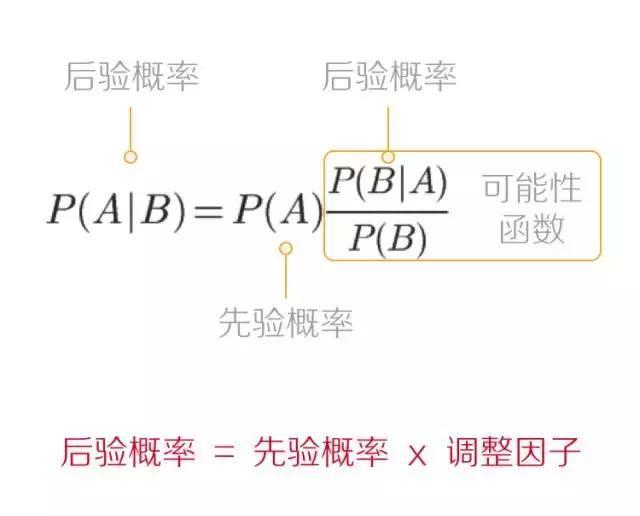## 0x2：一个贝叶斯推断的例子 - 图书管理员还是农民

### 1. 形式化定义这个问题

P(X|A)被定义为在史蒂文真的是一个图书管理员的情况下，史蒂文的邻居们给出的某种描述的概率，即如果史蒂文真的是一个图书管理员，他的邻居们将他描述为一个图书管理员的概率。这个值很可能接近于1，假设它为 0.95。

P(X) 可以解释为：任何人史蒂文的描述和史蒂文邻居的描述一致的概率，我们将其做逻辑上的改造：

P(X) = P(X and A) + P(X and ~A) = P(X|A)P(A) + P(X|~A)P(~A)

P(X|A)P(A) 这两个值我们都已经知道了。另外，~A 表示史蒂文不是一个图书管理员的概率，那么他一定是一个农民。所以 P(~A) = 1 - P(A) = 20 / 21。

P(X|~A) 即在史蒂文是一个农名的情况下，史蒂文的邻居给出的某个描述的概率，我们假设其为 0.5。

### 2. 通过可视化的代码呈现该问题

# -*- coding: utf-8 -*-
import numpy as np
from matplotlib import pyplot as plt

plt.rcParams['savefig.dpi'] = 300
plt.rcParams['figure.dpi'] = 300

colours = ["#348ABD", "#A60628"]

prior = [1/20., 20/21.]   # 图书管理员和农民的先验概率分别是 1/20 和 20/21
posterior = [0.087, 1-0.087]    # 后验概率
plt.bar([0, .7], prior, alpha=0.70, width=0.25,
color=colours, label="prior distribution",
lw="3", edgecolor=colours)

plt.bar([0 + 0.25, .7 + 0.25], posterior, alpha=0.7,
width=0.25, color=colours,
label="posterior distribution",
lw="3", edgecolor=colours)

plt.ylim(0, 1)
plt.xticks([0.20, 0.95], ['Librarian', 'Farmer'])
plt.title("Prior and Posterior probability of Steve's occupation")
plt.ylabel("Probability")
plt.legend(loc="upper left")

plt.show()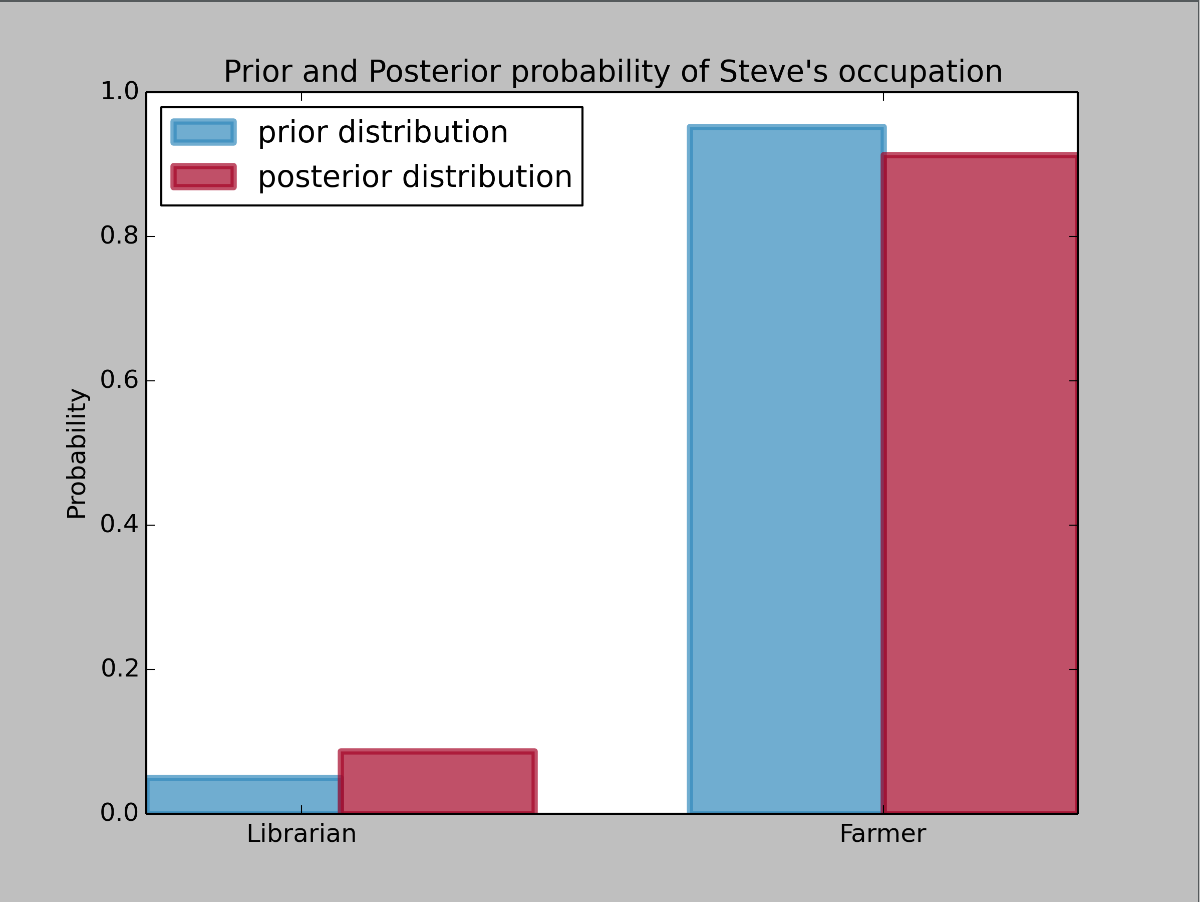# 3. 一个从短信数据推断行为模式的例子 - 使用计算机执行贝叶斯推断

## 0x1：场景描述 - 我们的数据是什么样的？

# -*- coding: utf-8 -*-
import numpy as np
from matplotlib import pyplot as plt

n_count_data = len(count_data)
plt.bar(np.arange(n_count_data), count_data, color="#348ABD")

plt.xlabel("Time (days)")
plt.title("Did the user's texting habits change over time?")
plt.xlim(0, n_count_data)

plt.show()## 0x3：使用什么生成式模型进行数据拟合？

### 2. 单位时间内随机事件发生的次数趋近于一个相对固定值 - 选泊松分布

1. 某医院平均每小时出生3个婴儿；
2. 某公司平均每10分钟接到1个电话；
3. 某超市平均每天销售4包 xx 牌奶粉；
4. 某网站平均每分钟有2次访问；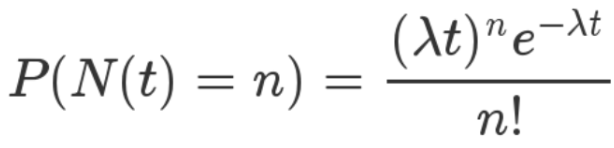P 表示概率，N 表示某种函数关系，t 表示时间，n 表示数量，λ 表示事件的频率。

1. 单位时间内发生 n 次数的概率随着时间 t 变化；

2. 概率受发生次数 n 的影响；

3. λ 相当于这件事的先验属性，在整个时间周期内都影响了最终的概率，λ 被称为此分布的一个超参数，它决定了这个分布的形式。

λ 可以位任意正数，随着 λ 的增加，得到大值的概率会增大。相反地，当 λ 减小时，得到小值的概率会增大。λ 可以被称为 Poisson分布的强度。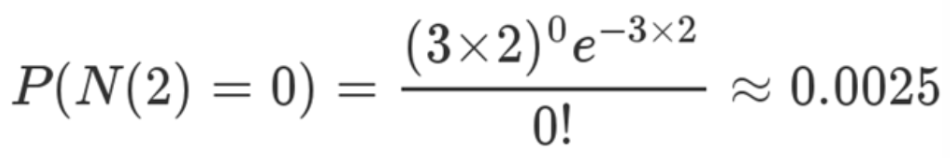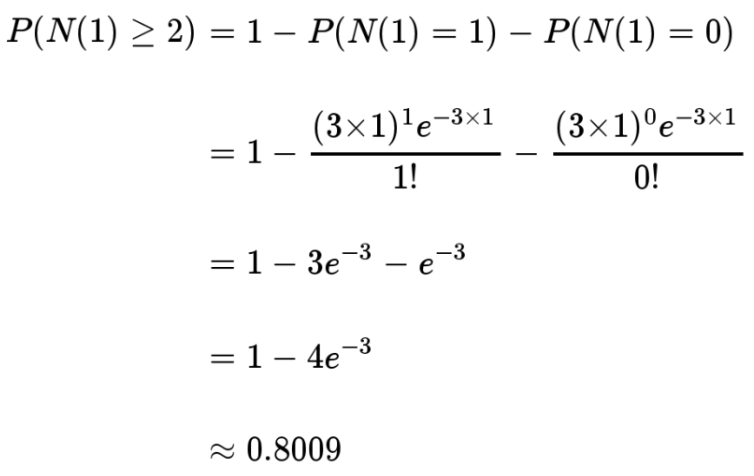Poisson分布的一个重要性质是：它的期望值等于它的参数。即：E[Z|λ] = λ。

# -*- coding: utf-8 -*-
import numpy as np
from matplotlib import pyplot as plt

import scipy.stats as stats
a = np.arange(16)
poi = stats.poisson
lambda_ = [1.5, 4.25]
colours = ["#348ABD", "#A60628"]

plt.bar(a, poi.pmf(a, lambda_), color=colours,
label="$\lambda = %.1f$" % lambda_, alpha=0.60,
edgecolor=colours, lw="3")

plt.bar(a, poi.pmf(a, lambda_), color=colours,
label="$\lambda = %.1f$" % lambda_, alpha=0.60,
edgecolor=colours, lw="3")

plt.xticks(a + 0.4, a)
plt.legend()
plt.ylabel("probability of $k$")
plt.xlabel("$k$")
plt.title("Probability mass function of a Poisson random variable; differing \
$\lambda$ values")

plt.show()### 3. 用单个泊松分布就足够拟合数据集了吗？### 4. 怎么形式化描述泊松分布参数 λ 的阶跃情况？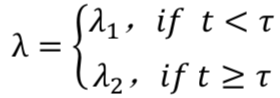1. 首先，存在一个 λ 阶跃点只是一个先验假设。事实上，可能并不存在这样一个 λ 阶跃点。
2. 第二，具体的阶跃点 τ 是什么时间呢？可能我们无法准确知道时间点，只能得到一个时间区间的概率分布。

#### 1）确定泊松分布参数 λ 的先验分布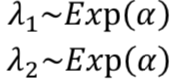α 称之为超参数。该参数能够影响其他参数。按照最大熵算法的原理，我们不希望对这个参数赋予太多的主观色彩。

#### 2）确定阶跃点 τ 的先验分布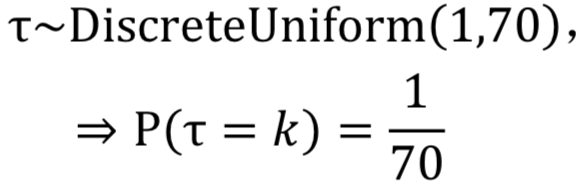## 0x4：使用PyMC进行贝叶斯推断

Cronin对概率编程有一段激动人心的描述：

换一种方式考虑这件事件，跟传统的编程仅仅向前运行不同的是，概率编程既可以向前也可以向后运行。它通过向前运行来计算其中包含的所有关于世界的假设结果（例如对模型空间的描述），但它也从数据中向后运行，以约束可能的解释。

### 1. 声明模型中的随机变量

# -*- coding: utf-8 -*-
import numpy as np
from matplotlib import pyplot as plt
import pymc as pm

n_count_data = len(count_data)
plt.bar(np.arange(n_count_data), count_data, color="#348ABD")
plt.xlabel("Time (days)")
plt.title("Did the user's texting habits change over time?")
plt.xlim(0, n_count_data)

alpha = 1.0 / count_data.mean()  # Recall count_data is the
# variable that holds our txt counts
lambda_1 = pm.Exponential("lambda_1", alpha)
lambda_2 = pm.Exponential("lambda_2", alpha)

tau = pm.DiscreteUniform("tau", lower=0, upper=n_count_data)

print("Random output:", tau.random(), tau.random(), tau.random())

### 2. 利用MCMC根据观测数据得到对模型参数的极大后验估计结果

# -*- coding: utf-8 -*-
import numpy as np
from matplotlib import pyplot as plt
import pymc as pm

n_count_data = len(count_data)
plt.bar(np.arange(n_count_data), count_data, color="#348ABD")
plt.xlabel("Time (days)")
plt.title("Did the user's texting habits change over time?")
plt.xlim(0, n_count_data)

alpha = 1.0 / count_data.mean()  # Recall count_data is the
# variable that holds our txt counts
lambda_1 = pm.Exponential("lambda_1", alpha)
lambda_2 = pm.Exponential("lambda_2", alpha)

tau = pm.DiscreteUniform("tau", lower=0, upper=n_count_data)

@pm.deterministic
def lambda_(tau=tau, lambda_1=lambda_1, lambda_2=lambda_2):
out = np.zeros(n_count_data)
out[:tau] = lambda_1  # lambda before tau is lambda1
out[tau:] = lambda_2  # lambda after (and including) tau is lambda2
return out

print("tau Random output:", tau.random(), tau.random(), tau.random())
#print("lambda distribution:", lambda_(tau, lambda_1, lambda_2))

observation = pm.Poisson("obs", lambda_, value=count_data, observed=True)

model = pm.Model([observation, lambda_1, lambda_2, tau])

mcmc = pm.MCMC(model)
mcmc.sample(40000, 10000, 1)

lambda_1_samples = mcmc.trace('lambda_1')[:]
lambda_2_samples = mcmc.trace('lambda_2')[:]
tau_samples = mcmc.trace('tau')[:]

# histogram of the samples:

ax = plt.subplot(311)
ax.set_autoscaley_on(False)

plt.hist(lambda_1_samples, histtype='stepfilled', bins=30, alpha=0.85,
label="posterior of $\lambda_1$", color="#A60628", normed=True)
plt.legend(loc="upper left")
plt.title(r"""Posterior distributions of the variables
$\lambda_1,\;\lambda_2,\;\tau$""")
plt.xlim([15, 30])
plt.xlabel("$\lambda_1$ value")

ax = plt.subplot(312)
ax.set_autoscaley_on(False)
plt.hist(lambda_2_samples, histtype='stepfilled', bins=30, alpha=0.85,
label="posterior of $\lambda_2$", color="#7A68A6", normed=True)
plt.legend(loc="upper left")
plt.xlim([15, 30])
plt.xlabel("$\lambda_2$ value")

plt.subplot(313)
w = 1.0 / tau_samples.shape * np.ones_like(tau_samples)
plt.hist(tau_samples, bins=n_count_data, alpha=1,
label=r"posterior of $\tau$",
color="#467821", weights=w, rwidth=2.)
plt.xticks(np.arange(n_count_data))

plt.legend(loc="upper left")
plt.ylim([0, .75])
plt.xlim([35, len(count_data) - 20])
plt.xlabel(r"$\tau$ (in days)")
plt.ylabel("probability")

plt.show()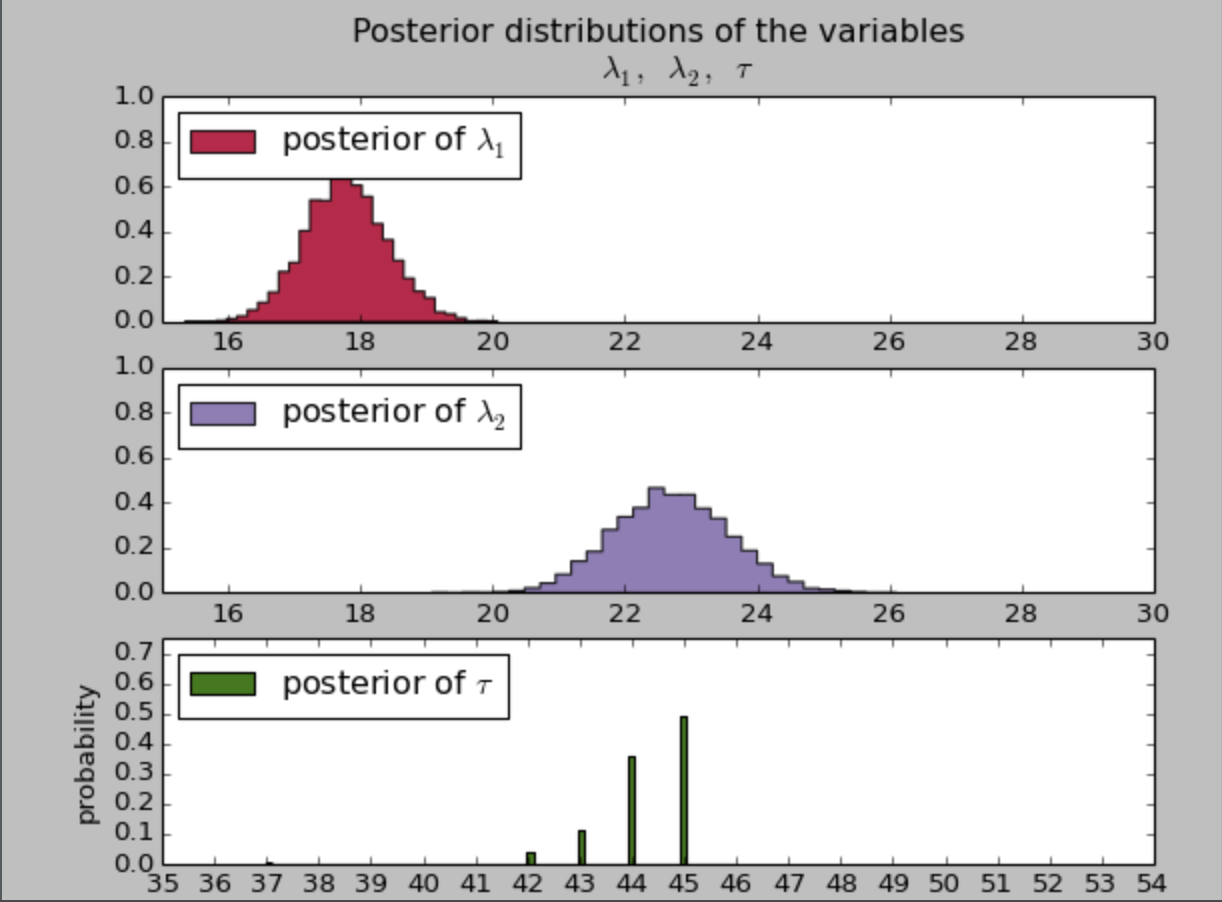## 0x6：根据模型参数得到后验样本

Poisson分布的期望值等于它的参数 λ。因此，问题相当于：在时间 t 中，参数 λ 的期望值是多少？

# -*- coding: utf-8 -*-
import numpy as np
from matplotlib import pyplot as plt
import pymc as pm

n_count_data = len(count_data)
plt.bar(np.arange(n_count_data), count_data, color="#348ABD")
plt.xlabel("Time (days)")
plt.title("Did the user's texting habits change over time?")
plt.xlim(0, n_count_data)

alpha = 1.0 / count_data.mean()  # Recall count_data is the
# variable that holds our txt counts
lambda_1 = pm.Exponential("lambda_1", alpha)
lambda_2 = pm.Exponential("lambda_2", alpha)

tau = pm.DiscreteUniform("tau", lower=0, upper=n_count_data)

@pm.deterministic
def lambda_(tau=tau, lambda_1=lambda_1, lambda_2=lambda_2):
out = np.zeros(n_count_data)
out[:tau] = lambda_1  # lambda before tau is lambda1
out[tau:] = lambda_2  # lambda after (and including) tau is lambda2
return out

print("tau Random output:", tau.random(), tau.random(), tau.random())
#print("lambda distribution:", lambda_(tau, lambda_1, lambda_2))

observation = pm.Poisson("obs", lambda_, value=count_data, observed=True)

model = pm.Model([observation, lambda_1, lambda_2, tau])

mcmc = pm.MCMC(model)
mcmc.sample(40000, 10000, 1)

lambda_1_samples = mcmc.trace('lambda_1')[:]
print "lambda_1_samples"
print lambda_1_samples

lambda_2_samples = mcmc.trace('lambda_2')[:]
print "lambda_2_samples"
print lambda_2_samples

tau_samples = mcmc.trace('tau')[:]
print "tau_samples"
print tau_samples

# tau_samples, lambda_1_samples, lambda_2_samples contain
# N samples from the corresponding posterior distribution
N = tau_samples.shape
expected_texts_per_day = np.zeros(n_count_data)
for day in range(0, n_count_data):
# ix is a bool index of all tau samples corresponding to
# the switchpoint occurring prior to value of 'day'
ix = day < tau_samples　　　　# 如果天数在转折点tau之前，取值lambda_1,否则取lambda_2,然后在取平均值
# Each posterior sample corresponds to a value for tau.
# for each day, that value of tau indicates whether we're "before"
# (in the lambda1 "regime") or
#  "after" (in the lambda2 "regime") the switchpoint.
# by taking the posterior sample of lambda1/2 accordingly, we can average
# over all samples to get an expected value for lambda on that day.
# As explained, the "message count" random variable is Poisson distributed,
# and therefore lambda (the poisson parameter) is the expected value of
# "message count".
print "lambda_1_samples[ix]"
print lambda_1_samples[ix]
print "lambda_2_samples[~ix]"
print lambda_2_samples[~ix]
expected_texts_per_day[day] = (lambda_1_samples[ix].sum()　　　　# 这个期望值我们假设是服从泊松分布的，分布的期望值＝参数lambda。
+ lambda_2_samples[~ix].sum()) / N

plt.plot(range(n_count_data), expected_texts_per_day, lw=4, color="#E24A33",
plt.xlim(0, n_count_data)
plt.xlabel("Day")
plt.ylabel("Expected # text-messages")
plt.ylim(0, 60)
plt.bar(np.arange(len(count_data)), count_data, color="#348ABD", alpha=0.65,
label="observed texts per day")

plt.legend(loc="upper left");

plt.show()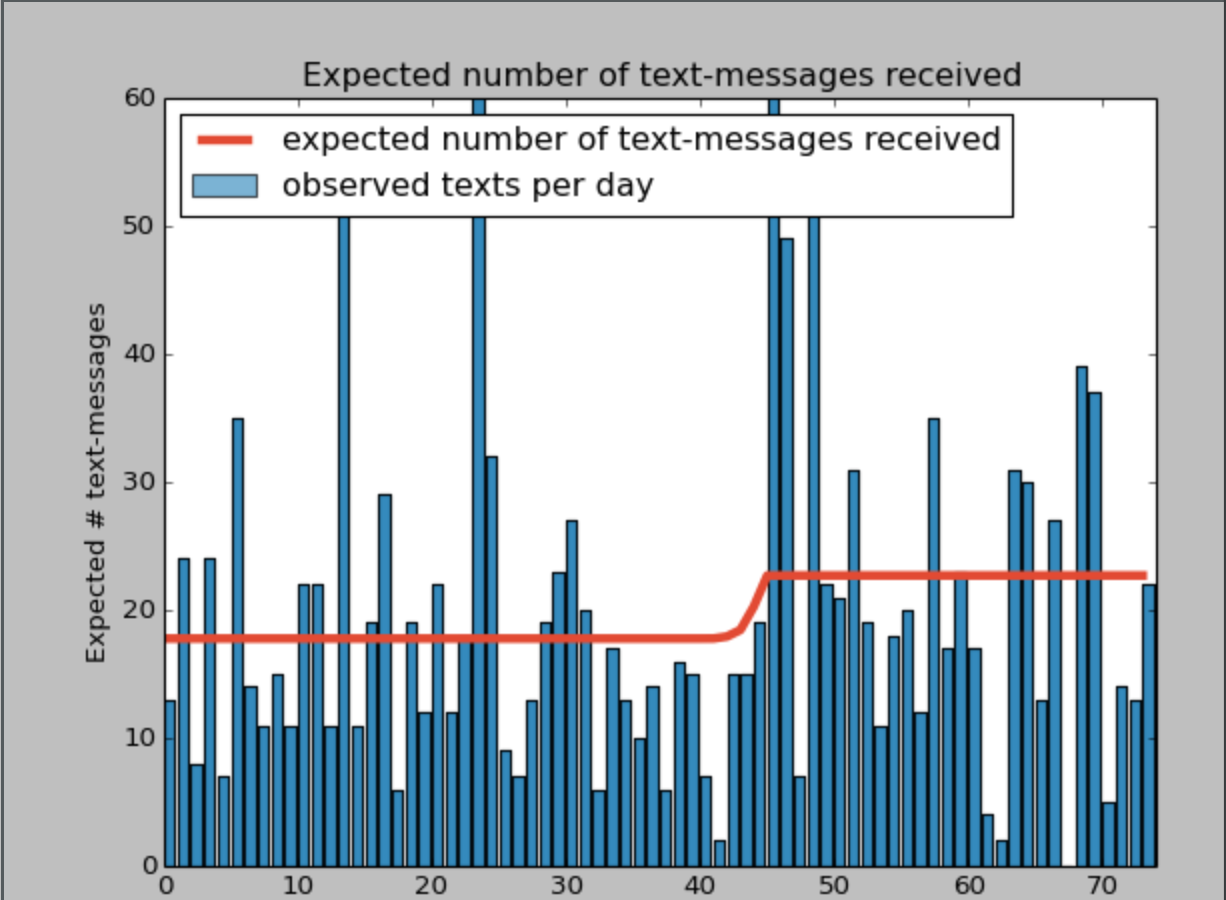### 1. 基于后验样本，从统计学上确定两个 λ 是否真的不一样

print (lambda_1_samples < lambda_2_samples).mean()

0.999933333333

## 0x7：扩充至两个阶跃点 - 能否借助贝叶斯推理自动挖掘潜在的规律？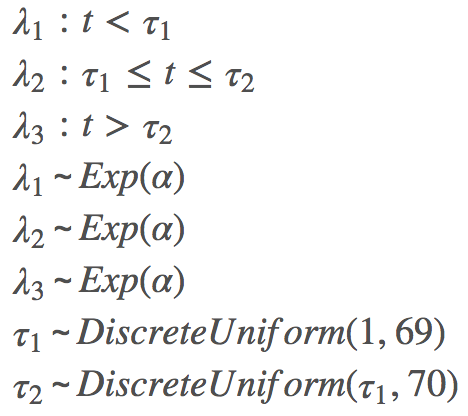# -*- coding: utf-8 -*-
import numpy as np
from matplotlib import pyplot as plt
import pymc as pm

n_count_data = len(count_data)
plt.bar(np.arange(n_count_data), count_data, color="#348ABD")
plt.xlabel("Time (days)")
plt.title("Did the user's texting habits change over time?")
plt.xlim(0, n_count_data)

alpha = 1.0 / count_data.mean()  # Recall count_data is the
# variable that holds our txt counts
lambda_1 = pm.Exponential("lambda_1", alpha)
lambda_2 = pm.Exponential("lambda_2", alpha)
lambda_3 = pm.Exponential("lambda_3", alpha)

tau_1 = pm.DiscreteUniform("tau_1", lower=0, upper=n_count_data-1)
tau_2 = pm.DiscreteUniform("tau_2", lower=tau_1, upper=n_count_data)

@pm.deterministic
def lambda_(tau_1=tau_1, tau_2=tau_2, lambda_1=lambda_1, lambda_2=lambda_2, lambda_3=lambda_3):
out = np.zeros(n_count_data)
out[:tau_1] = lambda_1  # lambda before tau is lambda1
out[tau_1:tau_2] = lambda_2  # lambda after (and including) tau is lambda2
out[tau_2:] = lambda_3  # lambda after (and including) tau is lambda2
return out

observation = pm.Poisson("obs", lambda_, value=count_data, observed=True)

model = pm.Model([observation, lambda_1, lambda_2, lambda_3, tau_1, tau_2])

mcmc = pm.MCMC(model)
mcmc.sample(40000, 10000)

lambda_1_samples = mcmc.trace('lambda_1')[:]
lambda_2_samples = mcmc.trace('lambda_2')[:]
lambda_3_samples = mcmc.trace('lambda_3')[:]
tau_1_samples = mcmc.trace('tau_1')[:]
tau_2_samples = mcmc.trace('tau_2')[:]

# histogram of the samples:

# lambda_1
ax = plt.subplot(311)
ax.set_autoscaley_on(False)
plt.hist(lambda_1_samples, histtype='stepfilled', bins=30,
label="$\lambda_1$", normed=True)
plt.legend(loc="upper left")
plt.grid(True)
plt.title(r"""Posterior distributions of the variables
$\lambda_1,\;\lambda_2,\;\tau$""")
# x轴坐标范围
plt.xlim([15, 30])
plt.xlabel("$\lambda_1$ value")

# lambda_2
ax = plt.subplot(312)
ax.set_autoscaley_on(False)
plt.hist(lambda_2_samples, histtype='stepfilled', bins=30,
label=" $\lambda_2$",color='#3009A6',normed=True)
plt.legend(loc="upper left")
plt.grid(True)
plt.xlim([30, 90])
plt.xlabel("$\lambda_2$ value")

# lambda_3
ax = plt.subplot(313)
ax.set_autoscaley_on(False)
plt.hist(lambda_3_samples, histtype='stepfilled', bins=30,
label=" $\lambda_2$",color='#6A63A6',normed=True)
plt.legend(loc="upper left")
plt.grid(True)
plt.xlim([15, 30])
plt.xlabel("$\lambda_3$ value")

plt.show()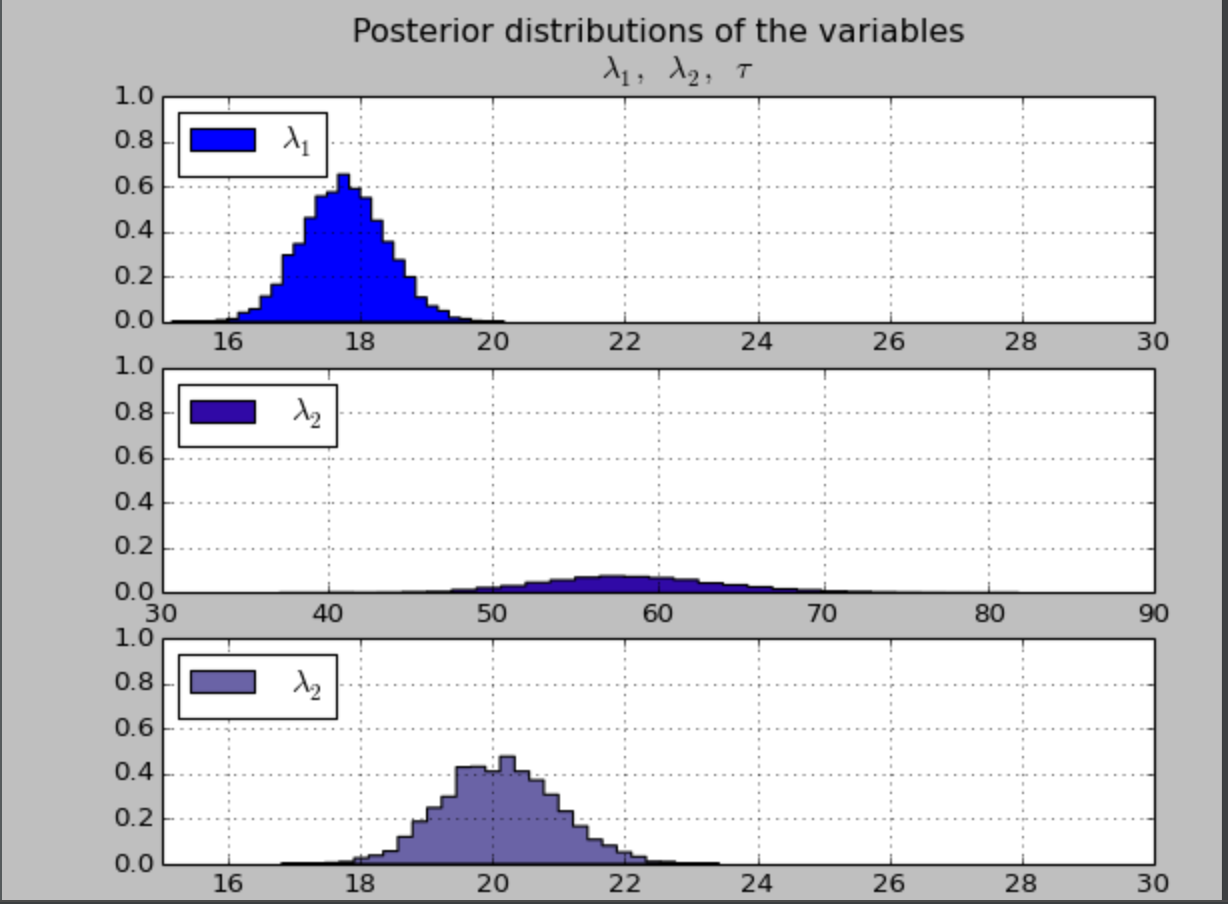# -*- coding: utf-8 -*-
import numpy as np
from matplotlib import pyplot as plt
import pymc as pm

n_count_data = len(count_data)
plt.bar(np.arange(n_count_data), count_data, color="#348ABD")
plt.xlabel("Time (days)")
plt.title("Did the user's texting habits change over time?")
plt.xlim(0, n_count_data)

alpha = 1.0 / count_data.mean()  # Recall count_data is the
# variable that holds our txt counts
lambda_1 = pm.Exponential("lambda_1", alpha)
lambda_2 = pm.Exponential("lambda_2", alpha)
lambda_3 = pm.Exponential("lambda_3", alpha)

tau_1 = pm.DiscreteUniform("tau_1", lower=0, upper=n_count_data-1)
tau_2 = pm.DiscreteUniform("tau_2", lower=tau_1, upper=n_count_data)

@pm.deterministic
def lambda_(tau_1=tau_1, tau_2=tau_2, lambda_1=lambda_1, lambda_2=lambda_2, lambda_3=lambda_3):
out = np.zeros(n_count_data)
out[:tau_1] = lambda_1  # lambda before tau is lambda1
out[tau_1:tau_2] = lambda_2  # lambda after (and including) tau is lambda2
out[tau_2:] = lambda_3  # lambda after (and including) tau is lambda2
return out

observation = pm.Poisson("obs", lambda_, value=count_data, observed=True)

model = pm.Model([observation, lambda_1, lambda_2, lambda_3, tau_1, tau_2])

mcmc = pm.MCMC(model)
mcmc.sample(40000, 10000)

lambda_1_samples = mcmc.trace('lambda_1')[:]
lambda_2_samples = mcmc.trace('lambda_2')[:]
lambda_3_samples = mcmc.trace('lambda_3')[:]
tau_1_samples = mcmc.trace('tau_1')[:]
tau_2_samples = mcmc.trace('tau_2')[:]

# histogram of the samples:

# lambda_1
# tau_1
w = 1.0 / tau_1_samples.shape * np.ones_like(tau_1_samples)
plt.hist(tau_1_samples, bins=count_data, alpha=1,
label=r"$\tau_1$",color="blue",weights=w )
plt.grid(True)
plt.legend(loc="upper left")
plt.xlabel(r"$\tau_1$ (in days)")
plt.ylabel("probability")

# tau_2
w = 1.0 / tau_2_samples.shape * np.ones_like(tau_1_samples)
plt.hist(tau_2_samples, bins=count_data, alpha=1,
label=r"$\tau_2$",weights=w,color="red",)
plt.xticks(np.arange(count_data))
plt.grid(True)
plt.legend(loc="upper left")
plt.ylim([0, 1.0])
plt.xlim([35, len(count_data) - 20])
plt.xlabel(r"$\tau_2$ (in days)")
plt.ylabel("probability")

plt.show()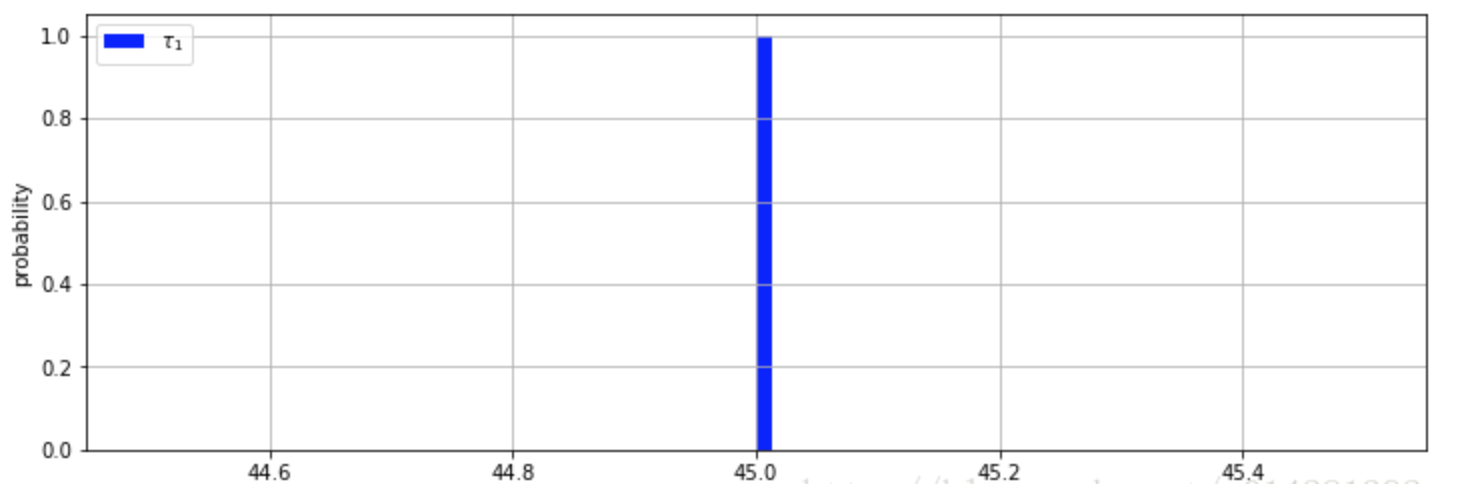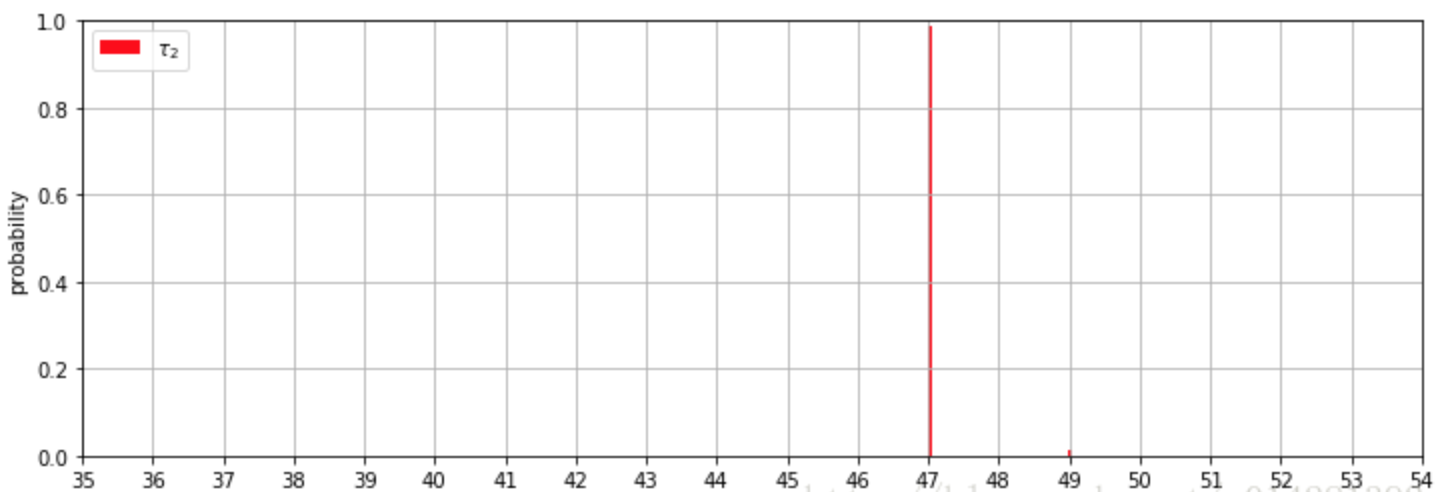https://blog.csdn.net/u014281392/article/details/79330286

# 4. 挑战者号事故案例

1986 年 1 月 28 日，美国航天飞机计划以灾难告终。在起飞后不就，航天飞机的其中一个助推器发生爆炸，七名宇航员全部遇难。总统的调查委员会得出的结论是，这场灾难是由于火箭推进器上的一个密封圈的失效引起的，导致失效的原因是密封圈对外界许多因素非常敏感，包括温度。

# -*- coding: utf-8 -*-
import numpy as np
from matplotlib import pyplot as plt

np.set_printoptions(precision=3, suppress=True)
usecols=[1, 2], missing_values="NA",
delimiter=",")
# drop the NA values
challenger_data = challenger_data[~np.isnan(challenger_data[:, 1])]

# plot it, as a function of temperature (the first column)
print("Temp (F), O-Ring failure?")
print(challenger_data)

plt.scatter(challenger_data[:, 0], challenger_data[:, 1], s=75, color="k",
alpha=0.5)
plt.yticks([0, 1])
plt.ylabel("Damage Incident?")
plt.xlabel("Outside temperature (Fahrenheit)")
plt.title("Defects of the Space Shuttle O-Rings vs temperature")

plt.show()

Date,Temperature,Damage Incident
04/12/1981,66,0
11/12/1981,70,1
3/22/82,69,0
6/27/82,80,NA
01/11/1982,68,0
04/04/1983,67,0
6/18/83,72,0
8/30/83,73,0
11/28/83,70,0
02/03/1984,57,1
04/06/1984,63,1
8/30/84,70,1
10/05/1984,78,0
11/08/1984,67,0
1/24/85,53,1
04/12/1985,67,0
4/29/85,75,0
6/17/85,70,0
7/29/85,81,0
8/27/85,76,0
10/03/1985,79,0
10/30/85,75,1
11/26/85,76,0
01/12/1986,58,1
1/28/86,31,Challenger Accident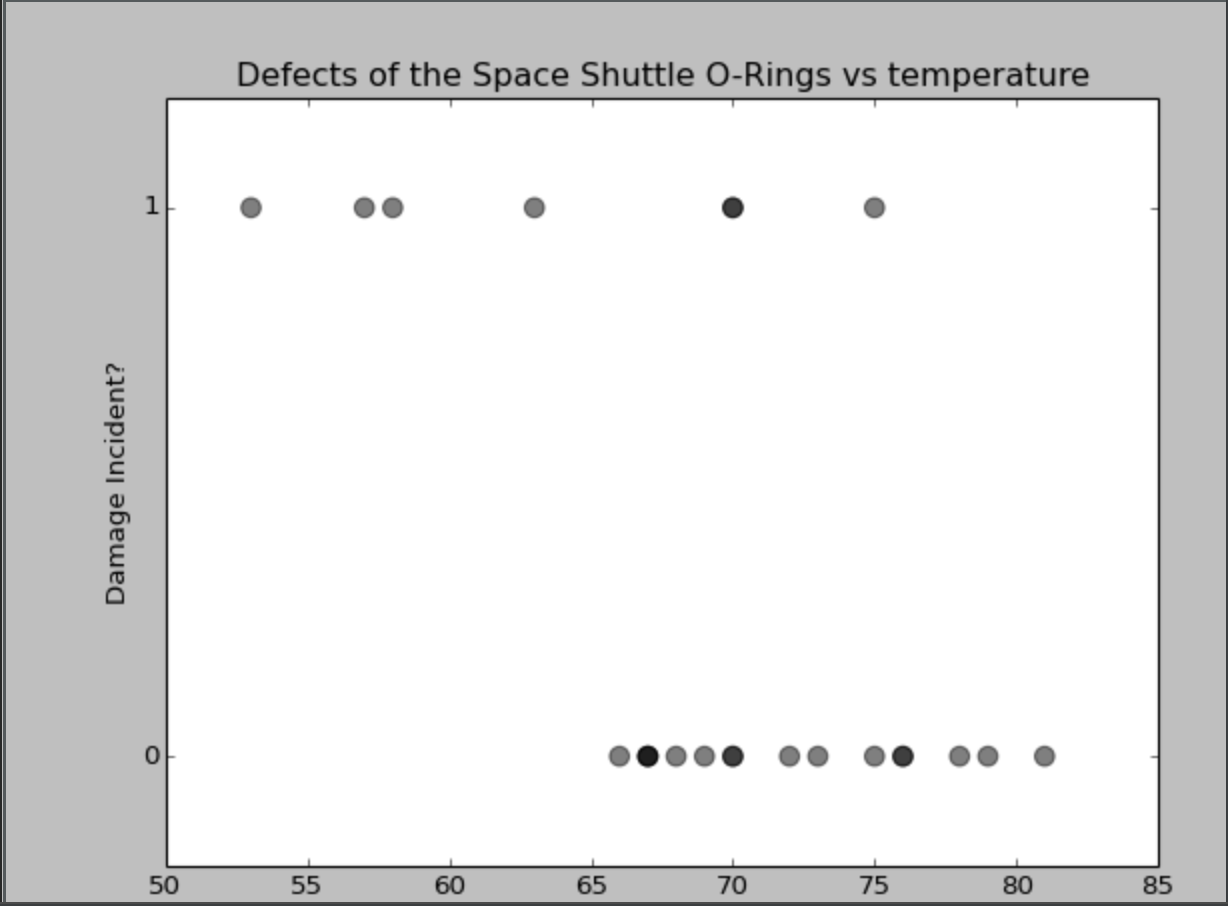## 0x1：我们如何对数据分布进行建模？

### 1. 通过非线性回归模拟样本数据的函数表示

# -*- coding: utf-8 -*-
# Code source: Gael Varoquaux

import numpy as np
import matplotlib.pyplot as plt

from sklearn import linear_model

# data set
X = np.array([
[66.],
[70.],
[69.],
[80.],
[68.],
[67.],
[72.],
[73.],
[70.],
[57.],
[63.],
[70.],
[78.],
[67.],
[53.],
[67.],
[75.],
[70.],
[81.],
[76.],
[79.],
[75.],
[76.],
[58.]
])

y = np.array([
0.,
1.,
0.,
0.,
0.,
0.,
0.,
0.,
0.,
1.,
1.,
1.,
0.,
0.,
1.,
0.,
0.,
0.,
0.,
0.,
0.,
1.,
0.,
1.
])

print "X"
print X.ravel()

print "y"
print y

# and plot the data
plt.figure(1, figsize=(8, 6))
plt.clf()
plt.scatter(X.ravel(), y, color='black', zorder=20)
X_test = np.linspace(50, 85, 300)

# run the classifier
clf = linear_model.LogisticRegression(C=1e5)
clf.fit(X, y)

def model(x):
y_value = 1 / (1 + np.exp(-x))
return y_value

# X_test * clf.coef_ + clf.intercept = 相当于: wx + b
# model()负责转换为逻辑斯蒂回归函数的 y 值
print "clf.coef: ", clf.coef_
print "clf.intercept_: ", clf.intercept_
y_value = model(X_test * clf.coef_ + clf.intercept_).ravel()
plt.plot(X_test, y_value, color='red', linewidth=3)     # 画出模型拟合后的曲线

plt.ylabel('y')
plt.xlabel('X')
plt.xticks(range(50, 85))
plt.yticks([0, 0.5, 1])
plt.ylim(-.25, 1.25)
plt.xlim(50, 85)
plt.legend(('Logistic Regression Model'),
loc="lower right", fontsize='small')
plt.show()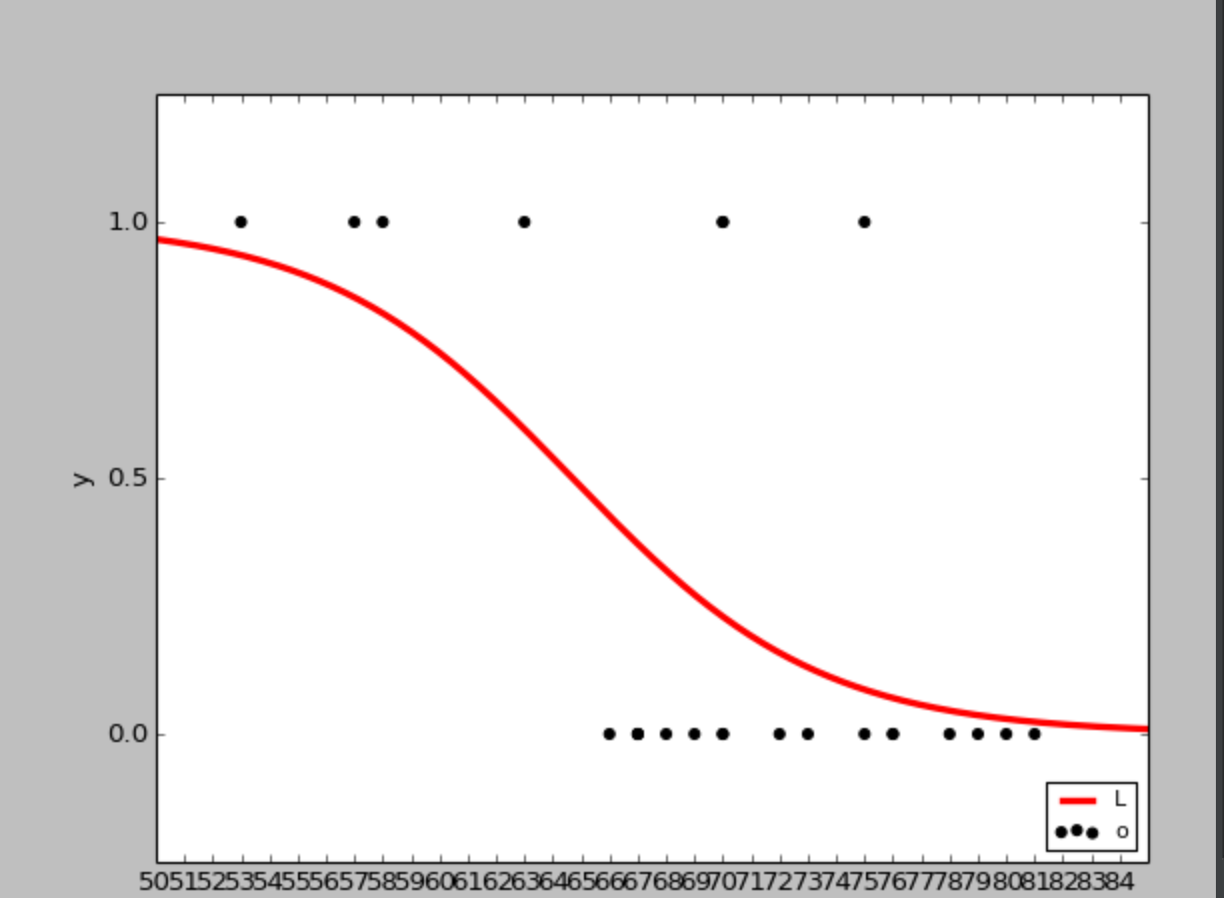clf.coef:  [[-0.22842526]]
clf.intercept_:  [ 14.77684766]

### 2. 通过数学建模和贝叶斯推理的方式进行数据拟合

#### 1）温度和事故发生的概率如何建模？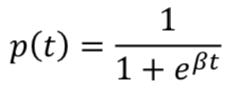𝛽 是这个模型的参数，是我们需要从数据中进行推理得到的。下面画出𝛽 = 1,3, −5时的𝑝(𝑡)的曲线

# -*- coding: utf-8 -*-

import numpy as np
import matplotlib.pyplot as plt

def logistic(x, beta):
return 1.0 / (1.0 + np.exp(beta * x))

x = np.linspace(-4, 4, 100)
plt.plot(x, logistic(x, 1), label=r"$\beta = 1$")
plt.plot(x, logistic(x, 3), label=r"$\beta = 3$")
plt.plot(x, logistic(x, -5), label=r"$\beta = -5$")
plt.legend()

plt.show()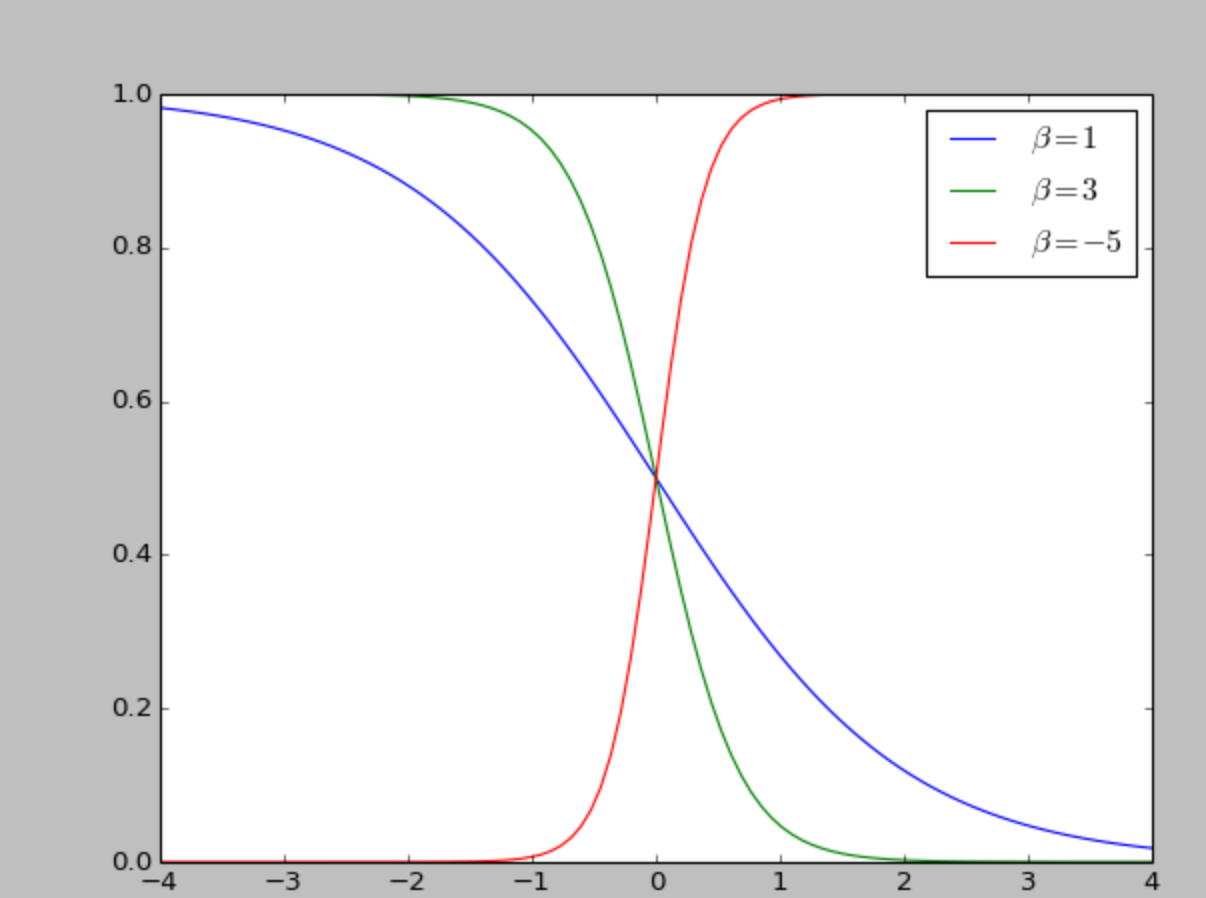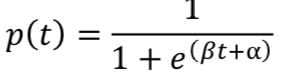# -*- coding: utf-8 -*-

import numpy as np
import matplotlib.pyplot as plt

def logistic(x, beta, alpha=0):
return 1.0 / (1.0 + np.exp(np.dot(beta, x) + alpha))

x = np.linspace(-4, 4, 100)
plt.plot(x, logistic(x, 1), label=r"$\beta = 1$", ls="--", lw=1)
plt.plot(x, logistic(x, 3), label=r"$\beta = 3$", ls="--", lw=1)
plt.plot(x, logistic(x, -5), label=r"$\beta = -5$", ls="--", lw=1)
plt.plot(x, logistic(x, 1, 1), label=r"$\beta = 1, \alpha = 1$",color="#348ABD")
plt.plot(x, logistic(x, 3, -2), label=r"$\beta = 3, \alpha = -2$",color="#A60628")
plt.plot(x, logistic(x, -5, 7), label=r"$\beta = -5, \alpha = 7$",color="#7A68A6")
plt.legend(loc="lower left");

plt.show()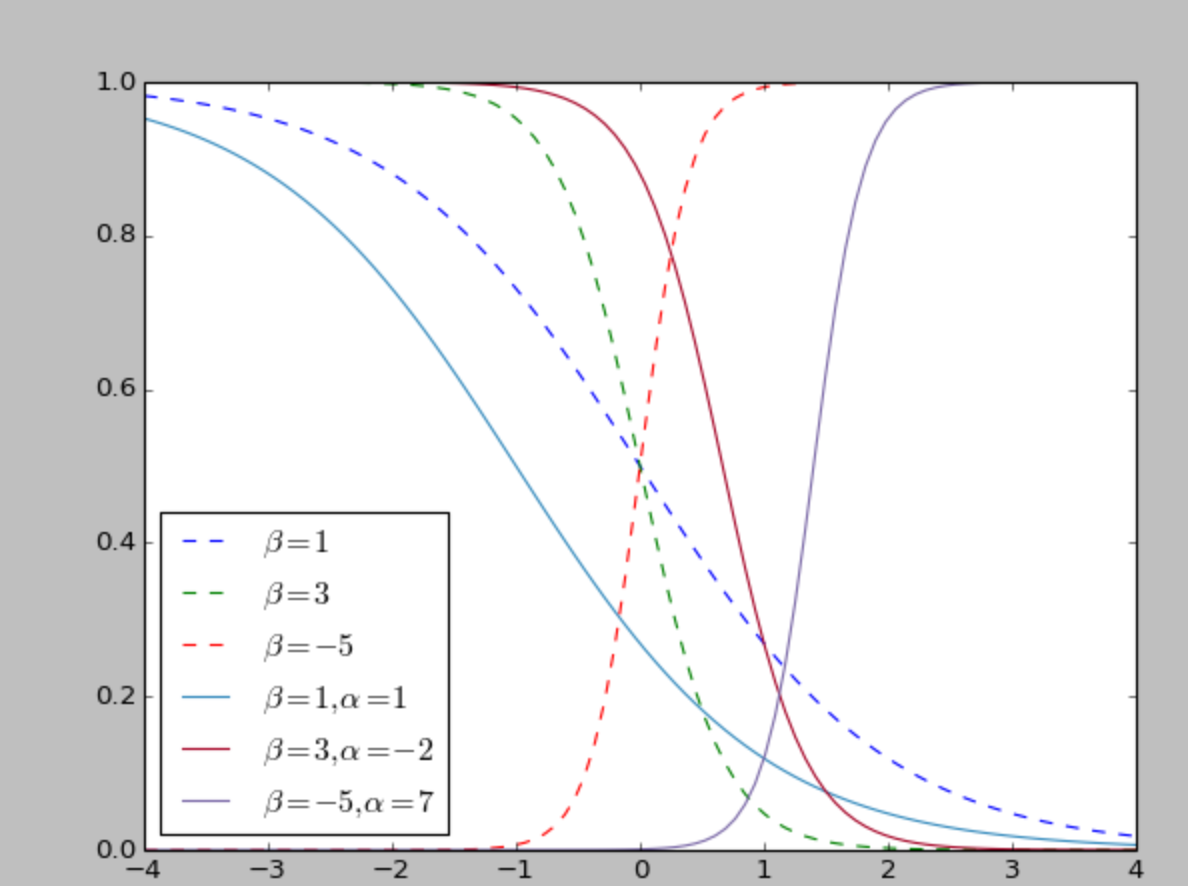𝛼 参数控制着曲线在横轴上左右移动，这也就是把 𝛼 称为偏置的原因。

#### 2）逻辑函数的参数如何建模？

import pymc as pm
temperature = challenger_data[:, 0]
D = challenger_data[:, 1] # defect or not?

# notice thevalue here. We explain why below. beta = pm.Normal("beta", 0, 0.001, value=0) alpha = pm.Normal("alpha", 0, 0.001, value=0) @pm.deterministic
def p(t=temperature, alpha=alpha, beta=beta):
return 1.0 / (1. + np.exp(beta * t + alpha))

#### 3）我们的观测数据如何和模型参数融合建模？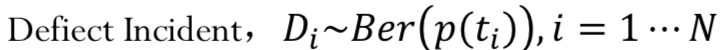，其中 p(t) 是我们的逻辑函数，ti 是观测到的温度值。

#### 4）通过pymc对观测样本进行模型参数的后验估计

# -*- coding: utf-8 -*-

import numpy as np
import matplotlib.pyplot as plt
import pymc as pm

np.set_printoptions(precision=3, suppress=True)
usecols=[1, 2], missing_values="NA",
delimiter=",")
# drop the NA values
challenger_data = challenger_data[~np.isnan(challenger_data[:, 1])]

# plot it, as a function of temperature (the first column)
print("Temp (F), O-Ring failure?")
print(challenger_data)

plt.scatter(challenger_data[:, 0], challenger_data[:, 1], s=75, color="k",
alpha=0.5)
plt.yticks([0, 1])
plt.ylabel("Damage Incident?")
plt.xlabel("Outside temperature (Fahrenheit)")
plt.title("Defects of the Space Shuttle O-Rings vs temperature")

temperature = challenger_data[:, 0]
D = challenger_data[:, 1]  # defect or not?

# notice thevalue here. We explain why below.
beta = pm.Normal("beta", 0, 0.001, value=0)
alpha = pm.Normal("alpha", 0, 0.001, value=0)

@pm.deterministic
def p(t=temperature, alpha=alpha, beta=beta):
return 1.0 / (1. + np.exp(beta * t + alpha))

# connect the probabilities in p with our observations through a
# Bernoulli random variable.
observed = pm.Bernoulli("bernoulli_obs", p, value=D, observed=True)

model = pm.Model([observed, beta, alpha])

# Mysterious code to be explained in Chapter 3
map_ = pm.MAP(model)
map_.fit()
mcmc = pm.MCMC(model)
mcmc.sample(120000, 100000, 2)

alpha_samples = mcmc.trace('alpha')[:, None]  # best to make them 1d
beta_samples = mcmc.trace('beta')[:, None]

# histogram of the samples:
plt.subplot(211)
plt.title(r"Posterior distributions of the variables $\alpha, \beta$")
plt.hist(beta_samples, histtype='stepfilled', bins=35, alpha=0.85,
label=r"posterior of $\beta$", color="#7A68A6", normed=True)
plt.legend()

plt.subplot(212)
plt.hist(alpha_samples, histtype='stepfilled', bins=35, alpha=0.85,
label=r"posterior of $\alpha$", color="#A60628", normed=True)
plt.legend()

plt.show()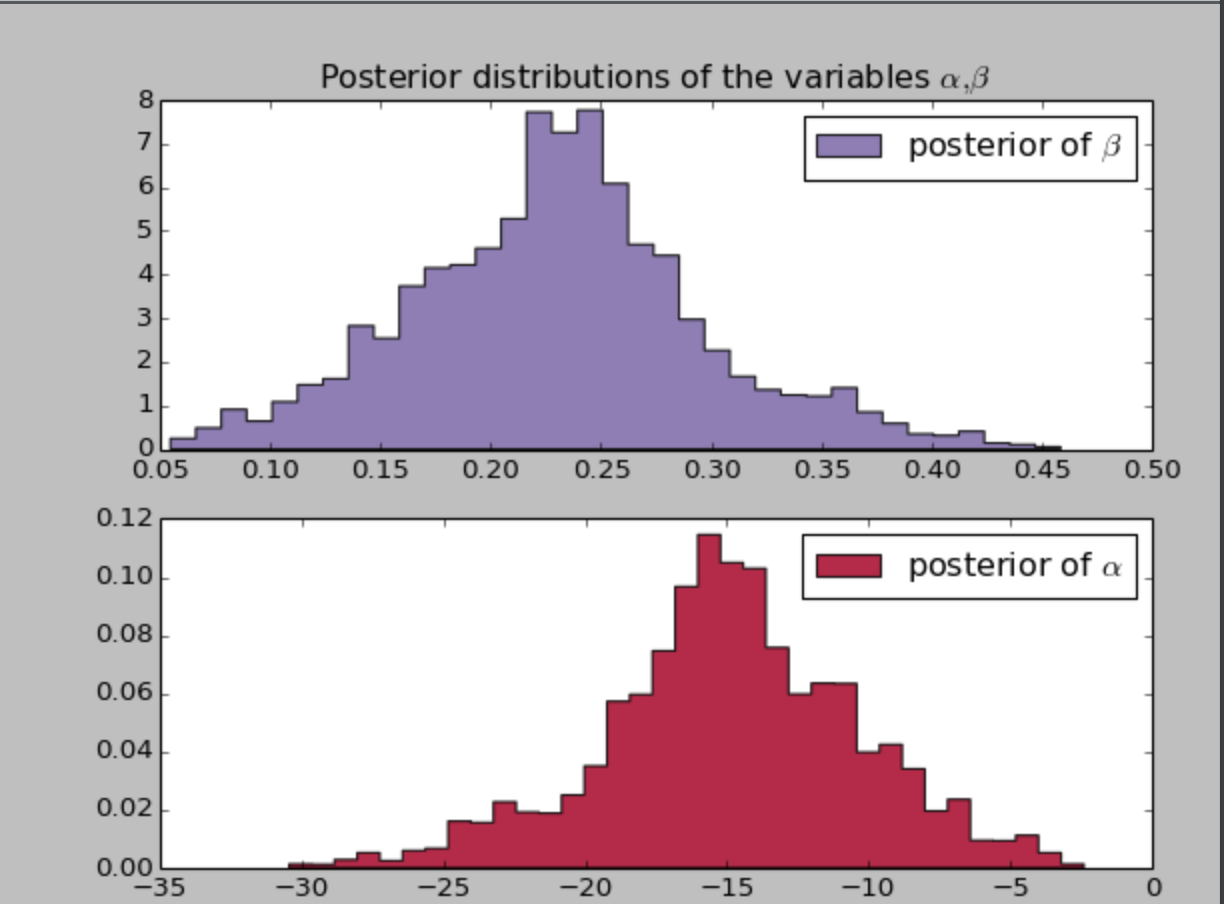## 0x3：基于模型参数的后验估计反推事件发生的概率的后验估计

# -*- coding: utf-8 -*-

import numpy as np
import matplotlib.pyplot as plt
import pymc as pm

np.set_printoptions(precision=3, suppress=True)
usecols=[1, 2], missing_values="NA",
delimiter=",")
# drop the NA values
challenger_data = challenger_data[~np.isnan(challenger_data[:, 1])]

# plot it, as a function of temperature (the first column)
print("Temp (F), O-Ring failure?")
print(challenger_data)

plt.scatter(challenger_data[:, 0], challenger_data[:, 1], s=75, color="k",
alpha=0.5)
plt.yticks([0, 1])
plt.ylabel("Damage Incident?")
plt.xlabel("Outside temperature (Fahrenheit)")
plt.title("Defects of the Space Shuttle O-Rings vs temperature")

temperature = challenger_data[:, 0]
D = challenger_data[:, 1]  # defect or not?

# notice thevalue here. We explain why below.
beta = pm.Normal("beta", 0, 0.001, value=0)
alpha = pm.Normal("alpha", 0, 0.001, value=0)

@pm.deterministic
def p(t=temperature, alpha=alpha, beta=beta):
return 1.0 / (1. + np.exp(beta * t + alpha))

def logistic(x, beta, alpha=0):
return 1.0 / (1.0 + np.exp(np.dot(beta, x) + alpha))

# connect the probabilities in p with our observations through a
# Bernoulli random variable.
observed = pm.Bernoulli("bernoulli_obs", p, value=D, observed=True)

model = pm.Model([observed, beta, alpha])

# Mysterious code to be explained in Chapter 3
map_ = pm.MAP(model)
map_.fit()
mcmc = pm.MCMC(model)
mcmc.sample(120000, 100000, 2)

alpha_samples = mcmc.trace('alpha')[:, None]  # best to make them 1d
beta_samples = mcmc.trace('beta')[:, None]

# 查看对既定的温度取值的期望概率，即我们队所有后验样本取均值，得到一个最大后验 p(t)
t = np.linspace(temperature.min() - 5, temperature.max() + 5, 50)[:, None]
p_t = logistic(t.T, beta_samples, alpha_samples)
print "p_t"
print p_t

mean_prob_t = p_t.mean(axis=0)

plt.plot(t, mean_prob_t, lw=3, label="average posterior \nprobability of defect")   # 求均值即求对特定温度的下事件发生的期望概率
plt.plot(t, p_t[0, :], ls="--", label="realization from posterior")     # 在不同的模型参数后验估计下，事件发生概率的后验估计
plt.plot(t, p_t[-2, :], ls="--", label="realization from posterior")    # 在不同的模型参数后验估计下，事件发生概率的后验估计
plt.scatter(temperature, D, color="k", s=50, alpha=0.5)
plt.title("Posterior expected value of probability of defect; \
plus realizations")
plt.legend(loc="lower left")
plt.ylim(-0.1, 1.1)
plt.xlim(t.min(), t.max())
plt.ylabel("probability")
plt.xlabel("temperature")

plt.show()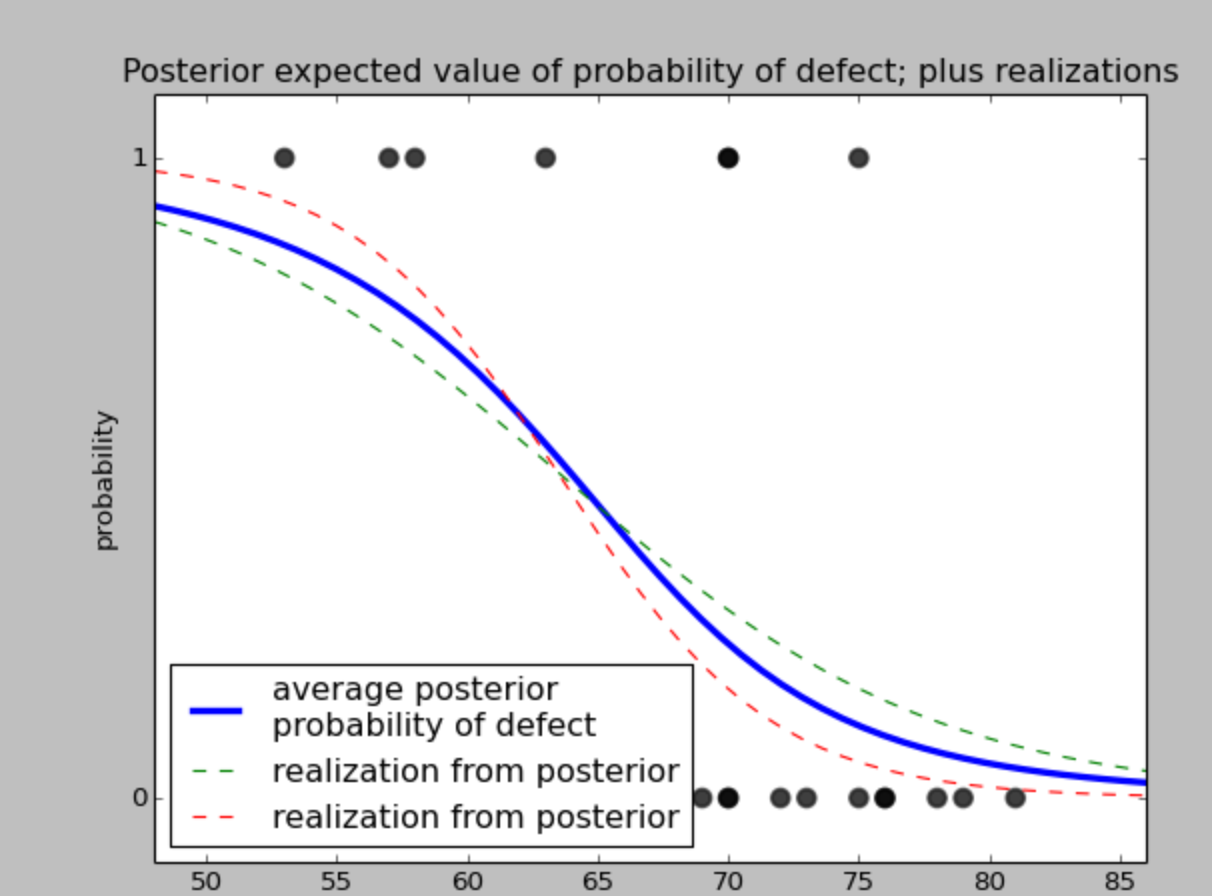### 1. 95%置信区间（CI）

# -*- coding: utf-8 -*-

import numpy as np
import matplotlib.pyplot as plt
import pymc as pm
from scipy.stats.mstats import mquantiles

np.set_printoptions(precision=3, suppress=True)
usecols=[1, 2], missing_values="NA",
delimiter=",")
# drop the NA values
challenger_data = challenger_data[~np.isnan(challenger_data[:, 1])]

# plot it, as a function of temperature (the first column)
print("Temp (F), O-Ring failure?")
print(challenger_data)

plt.scatter(challenger_data[:, 0], challenger_data[:, 1], s=75, color="k",
alpha=0.5)
plt.yticks([0, 1])
plt.ylabel("Damage Incident?")
plt.xlabel("Outside temperature (Fahrenheit)")
plt.title("Defects of the Space Shuttle O-Rings vs temperature")

temperature = challenger_data[:, 0]
D = challenger_data[:, 1]  # defect or not?

# notice thevalue here. We explain why below.
beta = pm.Normal("beta", 0, 0.001, value=0)
alpha = pm.Normal("alpha", 0, 0.001, value=0)

@pm.deterministic
def p(t=temperature, alpha=alpha, beta=beta):
return 1.0 / (1. + np.exp(beta * t + alpha))

def logistic(x, beta, alpha=0):
return 1.0 / (1.0 + np.exp(np.dot(beta, x) + alpha))

# connect the probabilities in p with our observations through a
# Bernoulli random variable.
observed = pm.Bernoulli("bernoulli_obs", p, value=D, observed=True)

model = pm.Model([observed, beta, alpha])

# Mysterious code to be explained in Chapter 3
map_ = pm.MAP(model)
map_.fit()
mcmc = pm.MCMC(model)
mcmc.sample(120000, 100000, 2)

alpha_samples = mcmc.trace('alpha')[:, None]  # best to make them 1d
beta_samples = mcmc.trace('beta')[:, None]

# 查看对既定的温度取值的期望概率，即我们队所有后验样本取均值，得到一个最大后验 p(t)
t = np.linspace(temperature.min() - 5, temperature.max() + 5, 50)[:, None]
p_t = logistic(t.T, beta_samples, alpha_samples)
print "p_t"
print p_t

mean_prob_t = p_t.mean(axis=0)

# vectorized bottom and top 2.5% quantiles for "confidence interval"
qs = mquantiles(p_t, [0.025, 0.975], axis=0)
plt.fill_between(t[:, 0], *qs, alpha=0.7,
color="#7A68A6")

plt.plot(t[:, 0], qs, label="95% CI", color="#7A68A6", alpha=0.7)

plt.plot(t, mean_prob_t, lw=1, ls="--", color="k",
label="average posterior \nprobability of defect")

plt.xlim(t.min(), t.max())
plt.ylim(-0.02, 1.02)
plt.legend(loc="lower left")
plt.scatter(temperature, D, color="k", s=50, alpha=0.5)
plt.xlabel("temp, $t$")

plt.ylabel("probability estimate")
plt.title("Posterior probability estimates given temp. $t$")

plt.show()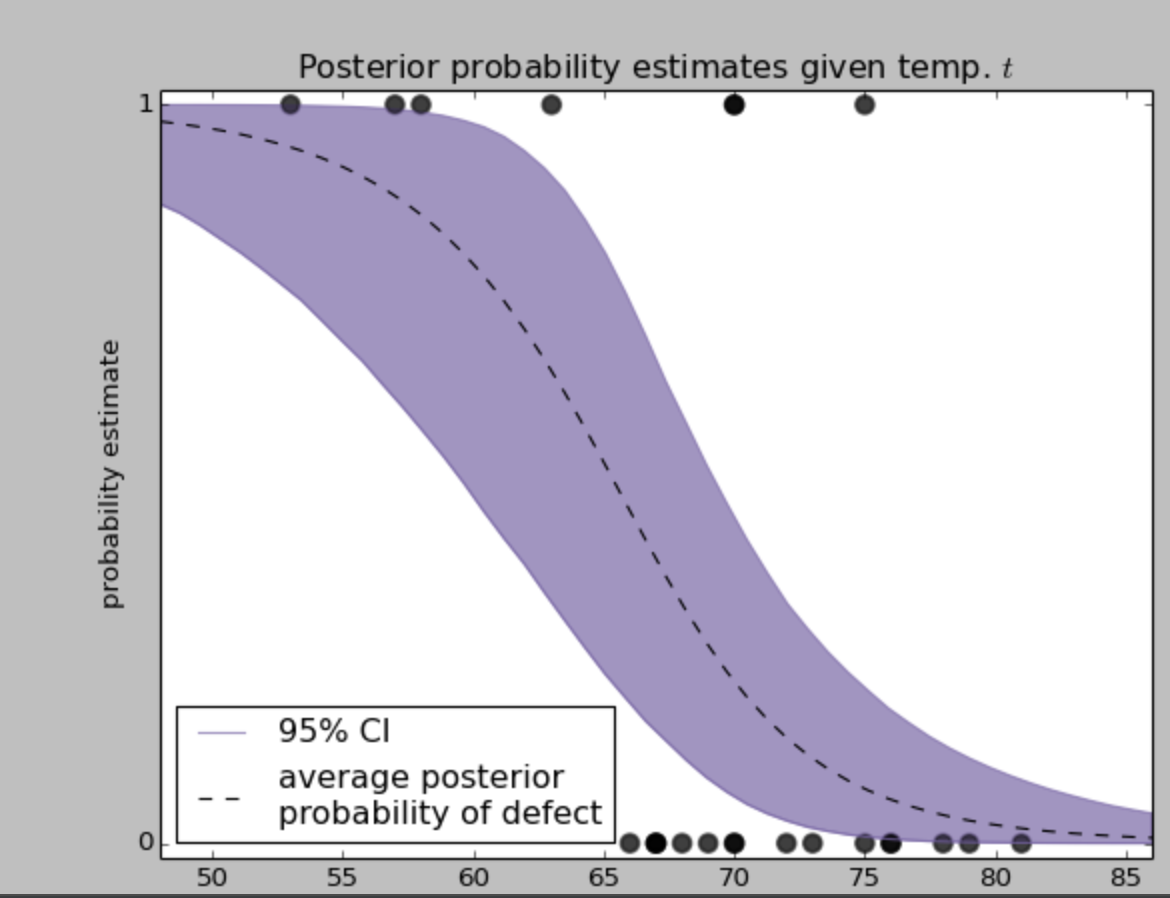95%置信区间，或者称95% CI，在图中用紫色区块显示。

1. 首先，在低温区间，尤其是50°以下，95%置信区间是很窄的，说明在低温时候发生事故的概率是非常大的，且这个事实非常确定；
2. 我们可能在温度区间 60-65 上做更多 的 O 形密封圈的测试，这样就能得到更好的估计概率。

### 2. 挑战者号事故当天发生了什么

# -*- coding: utf-8 -*-

import numpy as np
import matplotlib.pyplot as plt
import pymc as pm
from scipy.stats.mstats import mquantiles

np.set_printoptions(precision=3, suppress=True)
usecols=[1, 2], missing_values="NA",
delimiter=",")
# drop the NA values
challenger_data = challenger_data[~np.isnan(challenger_data[:, 1])]

# plot it, as a function of temperature (the first column)
print("Temp (F), O-Ring failure?")
print(challenger_data)

plt.scatter(challenger_data[:, 0], challenger_data[:, 1], s=75, color="k",
alpha=0.5)
plt.yticks([0, 1])
plt.ylabel("Damage Incident?")
plt.xlabel("Outside temperature (Fahrenheit)")
plt.title("Defects of the Space Shuttle O-Rings vs temperature")

temperature = challenger_data[:, 0]
D = challenger_data[:, 1]  # defect or not?

# notice thevalue here. We explain why below.
beta = pm.Normal("beta", 0, 0.001, value=0)
alpha = pm.Normal("alpha", 0, 0.001, value=0)

@pm.deterministic
def p(t=temperature, alpha=alpha, beta=beta):
return 1.0 / (1. + np.exp(beta * t + alpha))

def logistic(x, beta, alpha=0):
return 1.0 / (1.0 + np.exp(np.dot(beta, x) + alpha))

# connect the probabilities in p with our observations through a
# Bernoulli random variable.
observed = pm.Bernoulli("bernoulli_obs", p, value=D, observed=True)

model = pm.Model([observed, beta, alpha])

# Mysterious code to be explained in Chapter 3
map_ = pm.MAP(model)
map_.fit()
mcmc = pm.MCMC(model)
mcmc.sample(120000, 100000, 2)

alpha_samples = mcmc.trace('alpha')[:, None]  # best to make them 1d
beta_samples = mcmc.trace('beta')[:, None]

prob_31 = logistic(31, beta_samples, alpha_samples)

plt.xlim(0.995, 1)
plt.hist(prob_31, bins=1000, normed=True, histtype='stepfilled')
plt.title("Posterior distribution of probability of defect, given $t = 31$")
plt.xlabel("probability of defect occurring in O-ring");

plt.show()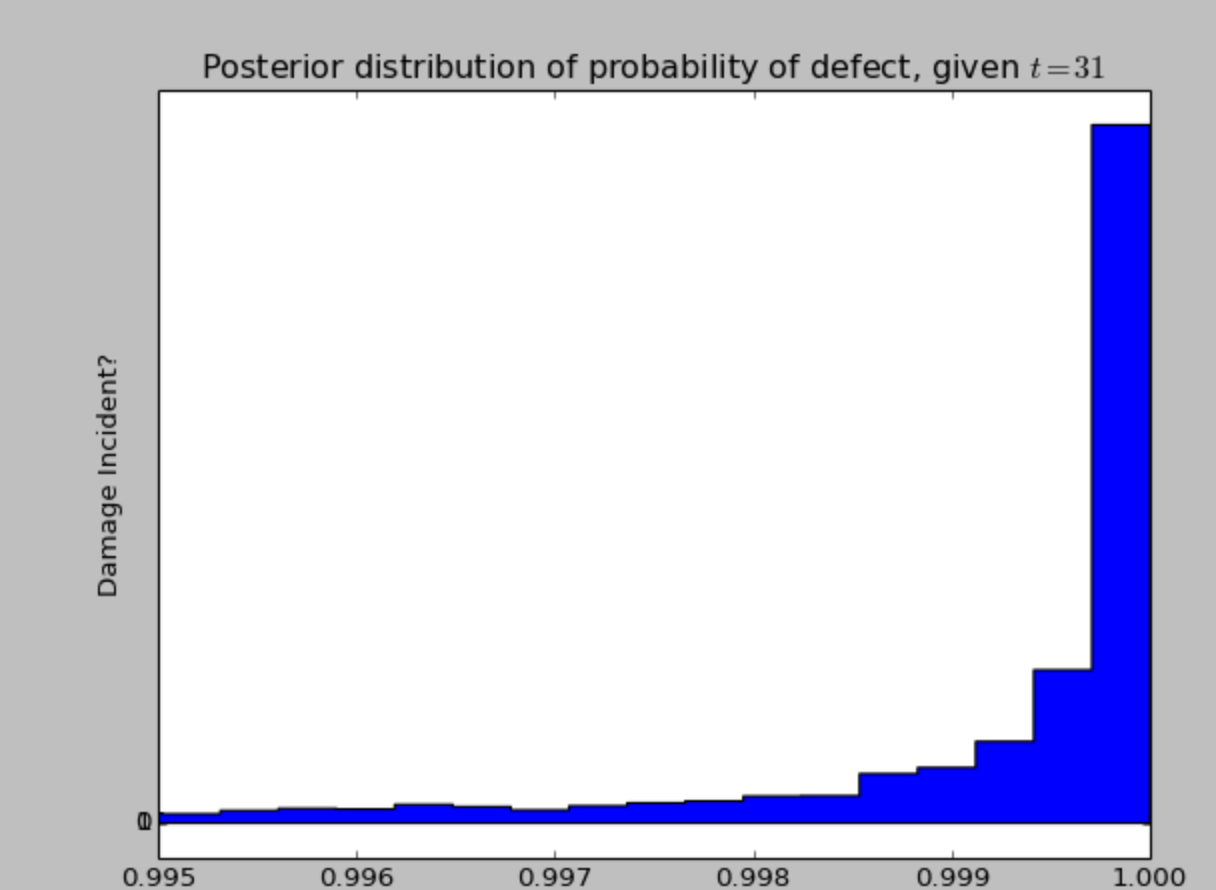## 0x4：我们的模型适用吗？

# -*- coding: utf-8 -*-

import numpy as np
import matplotlib.pyplot as plt
import pymc as pm
from scipy.stats.mstats import mquantiles

np.set_printoptions(precision=3, suppress=True)
usecols=[1, 2], missing_values="NA",
delimiter=",")
# drop the NA values
challenger_data = challenger_data[~np.isnan(challenger_data[:, 1])]

# plot it, as a function of temperature (the first column)
print("Temp (F), O-Ring failure?")
print(challenger_data)

plt.scatter(challenger_data[:, 0], challenger_data[:, 1], s=75, color="k",
alpha=0.5)
plt.yticks([0, 1])
plt.ylabel("Damage Incident?")
plt.xlabel("Outside temperature (Fahrenheit)")
plt.title("Defects of the Space Shuttle O-Rings vs temperature")

temperature = challenger_data[:, 0]
D = challenger_data[:, 1]  # defect or not?

# notice thevalue here. We explain why below.
beta = pm.Normal("beta", 0, 0.001, value=0)
alpha = pm.Normal("alpha", 0, 0.001, value=0)

@pm.deterministic
def p(t=temperature, alpha=alpha, beta=beta):
return 1.0 / (1. + np.exp(beta * t + alpha))

def logistic(x, beta, alpha=0):
return 1.0 / (1.0 + np.exp(np.dot(beta, x) + alpha))

# connect the probabilities in p with our observations through a
# Bernoulli random variable.
observed = pm.Bernoulli("bernoulli_obs", p, value=D, observed=True)

model = pm.Model([observed, beta, alpha])

# Mysterious code to be explained in Chapter 3
map_ = pm.MAP(model)
map_.fit()
mcmc = pm.MCMC(model)
mcmc.sample(120000, 100000, 2)

alpha_samples = mcmc.trace('alpha')[:, None]  # best to make them 1d
beta_samples = mcmc.trace('beta')[:, None]

simulated = pm.Bernoulli("bernoulli_sim", p)
N = 10000

mcmc = pm.MCMC([simulated, alpha, beta, observed])
mcmc.sample(N)

simulations = mcmc.trace("bernoulli_sim")[:]
print(simulations.shape)

plt.title("Simulated dataset using posterior parameters")

for i in range(4):
ax = plt.subplot(4, 1, i + 1)
plt.scatter(temperature, simulations[1000 * i, :], color="k",
s=50, alpha=0.6)

plt.show()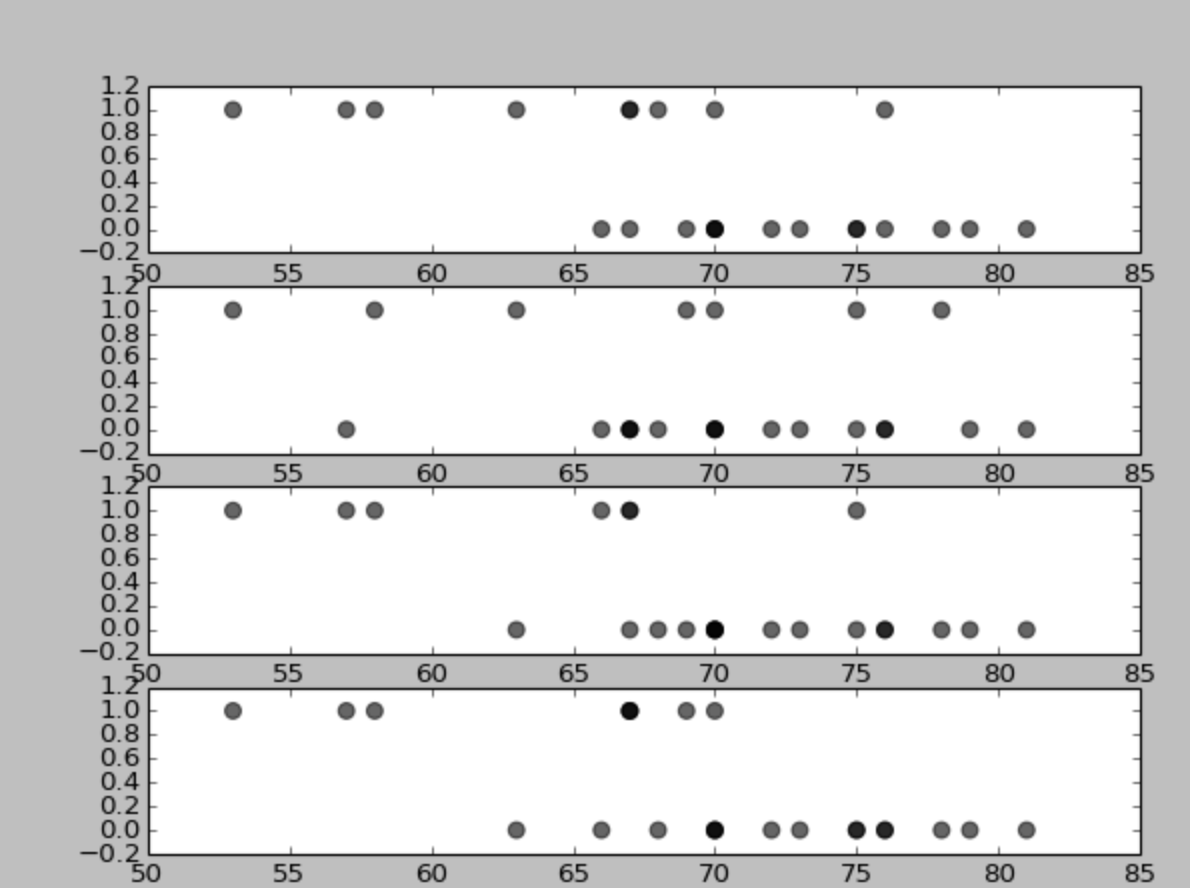### 1. 分离图

# -*- coding: utf-8 -*-

import numpy as np
import matplotlib.pyplot as plt
import pymc as pm
from scipy.stats.mstats import mquantiles

np.set_printoptions(precision=3, suppress=True)
usecols=[1, 2], missing_values="NA",
delimiter=",")
# drop the NA values
challenger_data = challenger_data[~np.isnan(challenger_data[:, 1])]

temperature = challenger_data[:, 0]
D = challenger_data[:, 1]  # defect or not?

# notice thevalue here. We explain why below.
beta = pm.Normal("beta", 0, 0.001, value=0)
alpha = pm.Normal("alpha", 0, 0.001, value=0)

@pm.deterministic
def p(t=temperature, alpha=alpha, beta=beta):
return 1.0 / (1. + np.exp(beta * t + alpha))

def logistic(x, beta, alpha=0):
return 1.0 / (1.0 + np.exp(np.dot(beta, x) + alpha))

# connect the probabilities in p with our observations through a
# Bernoulli random variable.
observed = pm.Bernoulli("bernoulli_obs", p, value=D, observed=True)

model = pm.Model([observed, beta, alpha])

# Mysterious code to be explained in Chapter 3
map_ = pm.MAP(model)
map_.fit()
mcmc = pm.MCMC(model)
mcmc.sample(120000, 100000, 2)

alpha_samples = mcmc.trace('alpha')[:, None]  # best to make them 1d
beta_samples = mcmc.trace('beta')[:, None]

simulated = pm.Bernoulli("bernoulli_sim", p)
N = 10000

mcmc = pm.MCMC([simulated, alpha, beta, observed])
mcmc.sample(N)

simulations = mcmc.trace("bernoulli_sim")[:]
print(simulations.shape)

# 对每一个模型，给定温度，计算后验模拟产生值1的次数比例，并对所有返回的模拟值取均值，这样我们得到在每一个数据点上发生缺陷的后验可能
posterior_probability = simulations.mean(axis=0)
print("posterior prob of defect | realized defect ")
for i in range(len(D)):
print("%.2f                     |   %d" % (posterior_probability[i], D[i]))

# 接下来我们根据后验概率对每一列进行排序
ix = np.argsort(posterior_probability)
print("probb | defect ")
for i in range(len(D)):
print("%.2f  |   %d" % (posterior_probability[ix[i]], D[ix[i]]))

# -*- coding: utf-8 -*-

import numpy as np
import matplotlib.pyplot as plt
import pymc as pm
from scipy.stats.mstats import mquantiles
from separation_plot import separation_plot

np.set_printoptions(precision=3, suppress=True)
usecols=[1, 2], missing_values="NA",
delimiter=",")
# drop the NA values
challenger_data = challenger_data[~np.isnan(challenger_data[:, 1])]

temperature = challenger_data[:, 0]
D = challenger_data[:, 1]  # defect or not?

# notice thevalue here. We explain why below.
beta = pm.Normal("beta", 0, 0.001, value=0)
alpha = pm.Normal("alpha", 0, 0.001, value=0)

@pm.deterministic
def p(t=temperature, alpha=alpha, beta=beta):
return 1.0 / (1. + np.exp(beta * t + alpha))

def logistic(x, beta, alpha=0):
return 1.0 / (1.0 + np.exp(np.dot(beta, x) + alpha))

# connect the probabilities in p with our observations through a
# Bernoulli random variable.
observed = pm.Bernoulli("bernoulli_obs", p, value=D, observed=True)

model = pm.Model([observed, beta, alpha])

# Mysterious code to be explained in Chapter 3
map_ = pm.MAP(model)
map_.fit()
mcmc = pm.MCMC(model)
mcmc.sample(120000, 100000, 2)

alpha_samples = mcmc.trace('alpha')[:, None]  # best to make them 1d
beta_samples = mcmc.trace('beta')[:, None]

simulated = pm.Bernoulli("bernoulli_sim", p)
N = 10000

mcmc = pm.MCMC([simulated, alpha, beta, observed])
mcmc.sample(N)

simulations = mcmc.trace("bernoulli_sim")[:]
print(simulations.shape)

# 对每一个模型，给定温度，计算后验模拟产生值1的次数比例，并对所有返回的模拟值取均值，这样我们得到在每一个数据点上发生缺陷的后验可能
posterior_probability = simulations.mean(axis=0)
# 可视化展示
separation_plot(posterior_probability, D)
plt.show()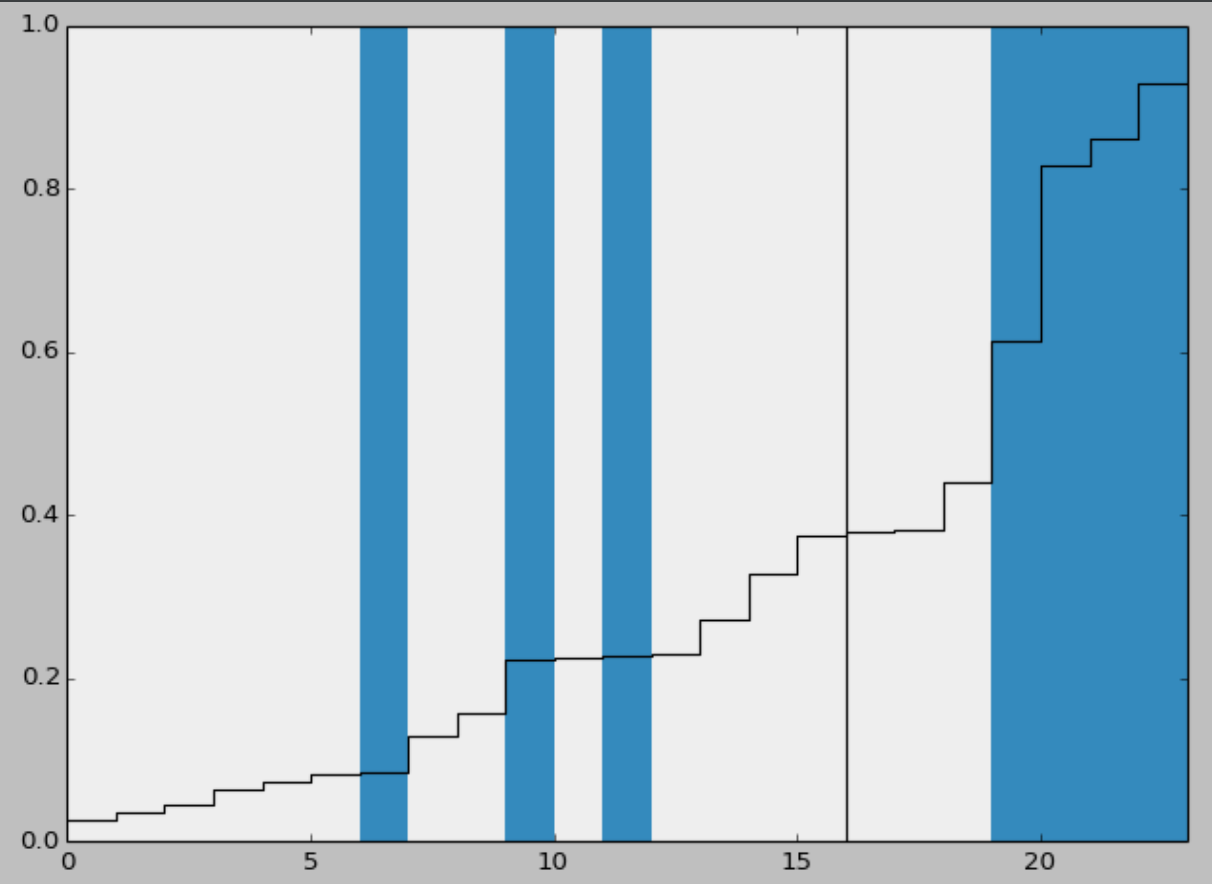# -*- coding: utf-8 -*-

import numpy as np
import matplotlib.pyplot as plt
import pymc as pm
from scipy.stats.mstats import mquantiles
from separation_plot import separation_plot

np.set_printoptions(precision=3, suppress=True)
usecols=[1, 2], missing_values="NA",
delimiter=",")
# drop the NA values
challenger_data = challenger_data[~np.isnan(challenger_data[:, 1])]

temperature = challenger_data[:, 0]
D = challenger_data[:, 1]  # defect or not?

# notice thevalue here. We explain why below.
beta = pm.Normal("beta", 0, 0.001, value=0)
alpha = pm.Normal("alpha", 0, 0.001, value=0)

@pm.deterministic
def p(t=temperature, alpha=alpha, beta=beta):
return 1.0 / (1. + np.exp(beta * t + alpha))

def logistic(x, beta, alpha=0):
return 1.0 / (1.0 + np.exp(np.dot(beta, x) + alpha))

# connect the probabilities in p with our observations through a
# Bernoulli random variable.
observed = pm.Bernoulli("bernoulli_obs", p, value=D, observed=True)

model = pm.Model([observed, beta, alpha])

# Mysterious code to be explained in Chapter 3
map_ = pm.MAP(model)
map_.fit()
mcmc = pm.MCMC(model)
mcmc.sample(120000, 100000, 2)

alpha_samples = mcmc.trace('alpha')[:, None]  # best to make them 1d
beta_samples = mcmc.trace('beta')[:, None]

simulated = pm.Bernoulli("bernoulli_sim", p)
N = 10000

mcmc = pm.MCMC([simulated, alpha, beta, observed])
mcmc.sample(N)

simulations = mcmc.trace("bernoulli_sim")[:]
print(simulations.shape)

# 对每一个模型，给定温度，计算后验模拟产生值1的次数比例，并对所有返回的模拟值取均值，这样我们得到在每一个数据点上发生缺陷的后验可能
posterior_probability = simulations.mean(axis=0)

# Our temperature-dependent model
separation_plot(posterior_probability, D)
plt.title("Temperature-dependent model")
plt.show()

# Perfect model
# i.e. the probability of defect is equal to if a defect occurred or not.
p = D
separation_plot(p, D)
plt.title("Perfect model")
plt.show()

# random predictions
p = np.random.rand(23)
separation_plot(p, D)
plt.title("Random model")
plt.show()

# constant model
constant_prob = 7. / 23 * np.ones(23)
separation_plot(constant_prob, D)
plt.title("Constant-prediction model")
plt.show()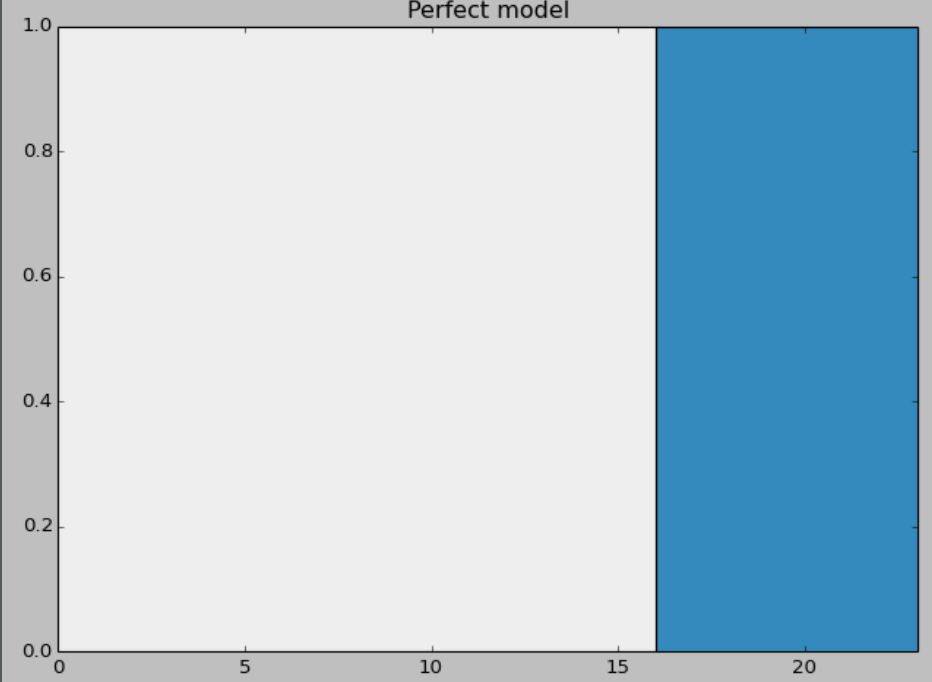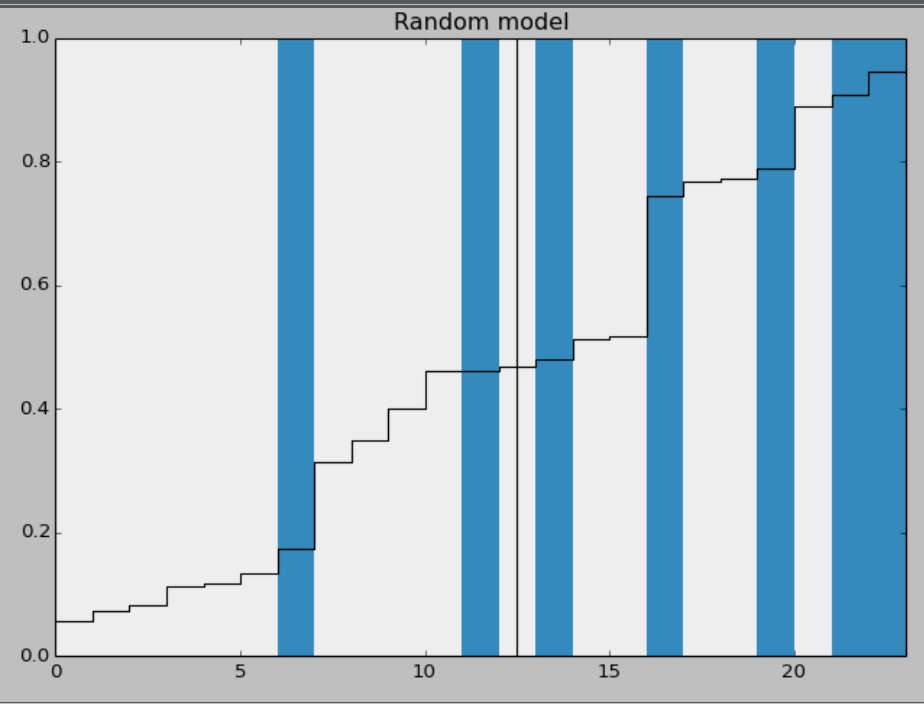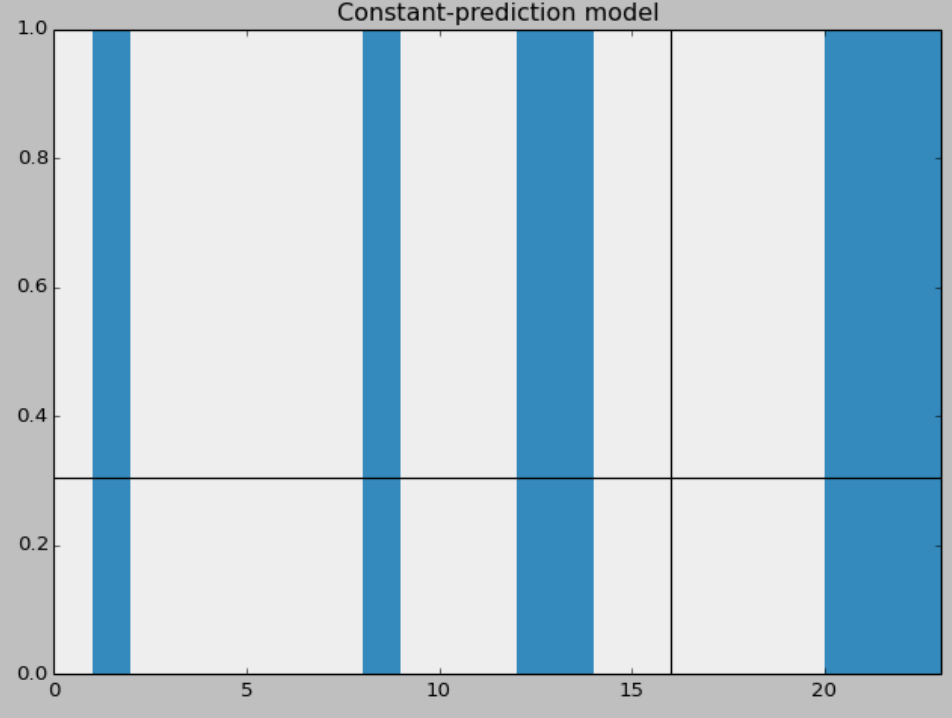1. 如果模型很完美，当事故确实发生时，它预测的后验概率是 1
2. 一个随机模型预测的结果完全是随机的，将和温度没有任何关系
3. 对于一个常数模型，即P(𝐷 = 1|𝑡) = 𝑐, ∀𝑡。𝑐的最优值是观测到的事故发生的概率，即𝑐 = 7⁄23

posted @ 2018-08-20 20:43  郑瀚Andrew.Hann  阅读(...)  评论(...编辑  收藏